Self-Portrait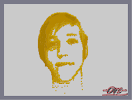Hover over the thumbnail for a full-size version.

Author Ors_II author:ors_ii n-art n-artist ors program rated self-portrait 2014-10-05 2019-02-28 4 by 11 people. \$Self-Portrait#Ors_II#self-portrait#00000000000000000000000000000000000000000000000000000000000000000000000000000000000000000000000000000000000000000000000000000000000000000000000000000000000000000000000000000000000000000000000000000000000000000000000000000000000000000000000000000000000000000000000000000000000000000000000000000000000000000000000000000000000000000000000000000000000000000000000000000000000000000000000000000000000000000000000000000000000000000000000000000000000000000000000000000000000000000000000000000000000000000000000000000000000000000000000000000000000000000000000000000000000000000000000000000000000000000000000000000000000000000000000000000000000000000000000000000000000000000000000000000000000000000000000000000000000000000|3^619,571!3^620,571!3^621,571!3^622,571!3^623,571!3^624,571!3^625,571!3^626,571!3^703,571!3^704,571!3^705,571!3^706,571!3^707,571!3^708,571!3^709,571!3^710,571!3^711,571!3^712,571!3^713,571!3^714,571!3^715,571!3^716,571!3^717,571!3^718,571!3^719,571!3^720,571!3^721,571!3^722,571!3^723,571!3^724,571!3^725,571!3^726,571!3^727,571!12^618,570!12^619,570!12^620,570!12^621,570!12^622,570!12^623,570!12^624,570!12^625,570!3^626,570!3^627,570!3^628,570!3^629,570!3^630,570!3^631,570!3^632,570!3^633,570!3^634,570!3^635,570!3^636,570!3^637,570!3^638,570!3^686,570!3^687,570!3^688,570!3^689,570!3^690,570!3^691,570!3^692,570!3^693,570!3^694,570!3^695,570!3^696,570!3^697,570!3^698,570!3^699,570!3^700,570!3^701,570!12^702,570!12^703,570!12^704,570!12^705,570!12^706,570!12^707,570!12^708,570!12^709,570!12^710,570!12^711,570!12^712,570!12^713,570!12^714,570!12^715,570!12^716,570!12^717,570!12^718,570!12^719,570!12^720,570!12^721,570!12^722,570!12^723,570!12^724,570!12^725,570!12^726,570!3^727,570!3^728,570!3^729,570!3^730,570!3^731,570!3^732,570!3^733,570!3^734,570!3^735,570!3^736,570!3^737,570!3^738,570!12^617,569!12^618,569!12^619,569!12^620,569!12^621,569!12^622,569!12^623,569!12^624,569!12^625,569!12^626,569!12^627,569!12^628,569!12^629,569!12^630,569!12^631,569!12^632,569!12^633,569!12^634,569!12^635,569!12^636,569!12^637,569!3^638,569!3^639,569!3^640,569!3^641,569!3^642,569!3^643,569!3^644,569!3^645,569!3^646,569!3^647,569!3^648,569!3^649,569!3^650,569!3^651,569!3^652,569!3^653,569!3^654,569!3^655,569!3^656,569!3^657,569!3^658,569!3^659,569!3^660,569!3^661,569!3^662,569!3^663,569!3^664,569!3^665,569!3^666,569!3^667,569!3^668,569!3^669,569!3^670,569!3^671,569!3^672,569!3^673,569!3^674,569!3^675,569!3^676,569!3^677,569!3^678,569!3^679,569!3^680,569!3^681,569!3^682,569!3^683,569!3^684,569!12^685,569!12^686,569!12^687,569!12^688,569!12^689,569!12^690,569!12^691,569!12^692,569!12^693,569!12^694,569!12^695,569!12^696,569!12^697,569!12^698,569!12^699,569!12^700,569!12^701,569!12^702,569!12^703,569!12^704,569!12^705,569!12^706,569!12^707,569!12^708,569!12^709,569!12^710,569!12^711,569!12^712,569!12^713,569!12^714,569!12^715,569!12^716,569!12^717,569!12^718,569!12^719,569!12^720,569!12^721,569!12^722,569!12^723,569!12^724,569!12^725,569!12^726,569!12^727,569!12^728,569!12^729,569!12^730,569!12^731,569!12^732,569!12^733,569!12^734,569!12^735,569!12^736,569!12^737,569!3^738,569!3^739,569!3^740,569!3^741,569!3^742,569!3^743,569!3^744,569!3^745,569!3^746,569!3^747,569!12^626,568!12^627,568!12^628,568!12^629,568!12^630,568!12^631,568!12^632,568!12^633,568!12^634,568!12^635,568!12^636,568!12^637,568!12^638,568!12^639,568!12^640,568!12^641,568!12^642,568!12^643,568!12^644,568!12^645,568!12^646,568!12^647,568!12^648,568!12^649,568!12^650,568!12^651,568!12^652,568!12^653,568!12^654,568!12^655,568!12^656,568!12^657,568!12^658,568!12^659,568!12^660,568!12^661,568!12^662,568!12^663,568!12^664,568!12^665,568!12^666,568!12^667,568!12^668,568!12^669,568!12^670,568!12^671,568!12^672,568!12^673,568!12^674,568!12^675,568!12^676,568!12^677,568!12^678,568!12^679,568!12^680,568!12^681,568!12^682,568!12^683,568!12^684,568!12^685,568!12^686,568!12^687,568!12^688,568!12^689,568!12^690,568!12^691,568!12^692,568!12^693,568!12^694,568!12^695,568!12^696,568!12^697,568!12^698,568!12^699,568!12^700,568!12^701,568!12^726,568!12^727,568!12^728,568!12^729,568!12^730,568!12^731,568!12^732,568!12^733,568!12^734,568!12^735,568!12^736,568!12^737,568!12^738,568!12^739,568!12^740,568!12^741,568!12^742,568!12^743,568!12^744,568!12^745,568!12^746,568!3^747,568!3^748,568!3^749,568!3^750,568!3^751,568!3^752,568!3^753,568!3^754,568!12^638,567!12^639,567!12^640,567!12^641,567!12^642,567!12^643,567!12^644,567!12^645,567!12^646,567!12^647,567!12^648,567!12^649,567!12^650,567!12^651,567!12^652,567!12^653,567!12^654,567!12^655,567!12^656,567!12^657,567!12^658,567!12^659,567!12^660,567!12^661,567!12^662,567!12^663,567!12^664,567!12^665,567!12^666,567!12^667,567!12^668,567!12^669,567!12^670,567!12^671,567!12^672,567!12^673,567!12^674,567!12^675,567!12^676,567!12^677,567!12^678,567!12^679,567!12^680,567!12^681,567!12^682,567!12^683,567!12^684,567!12^739,567!12^740,567!12^741,567!12^742,567!12^743,567!12^744,567!12^745,567!12^746,567!12^747,567!12^748,567!12^749,567!12^750,567!12^751,567!12^752,567!12^753,567!3^754,567!3^755,567!3^756,567!3^757,567!3^758,567!3^759,567!3^760,567!3^761,567!12^747,566!12^748,566!12^749,566!12^750,566!12^751,566!12^752,566!12^753,566!12^754,566!12^755,566!12^756,566!12^757,566!12^758,566!12^759,566!12^760,566!3^761,566!3^762,566!3^763,566!12^754,565!12^755,565!12^756,565!12^757,565!12^758,565!12^759,565!12^760,565!12^761,565!12^762,565!3^763,565!12^761,564!12^762,564!0^388,559!3^650,557!3^651,557!3^652,557!3^653,557!3^648,556!12^649,556!12^650,556!12^651,556!12^652,556!3^653,556!3^654,556!3^655,556!3^656,556!3^657,556!3^658,556!3^659,556!3^684,556!3^685,556!3^686,556!3^705,556!3^706,556!3^707,556!3^708,556!3^709,556!3^710,556!3^711,556!3^712,556!3^713,556!12^647,555!12^648,555!12^649,555!12^650,555!12^651,555!12^652,555!12^653,555!12^654,555!12^655,555!12^656,555!12^657,555!12^658,555!3^659,555!3^660,555!3^661,555!12^683,555!12^684,555!12^685,555!3^686,555!3^687,555!12^704,555!12^705,555!12^706,555!12^707,555!12^708,555!12^709,555!12^710,555!12^711,555!12^712,555!3^713,555!3^714,555!3^715,555!3^716,555!3^717,555!12^646,554!12^647,554!12^648,554!12^649,554!12^650,554!12^651,554!12^652,554!12^653,554!12^654,554!12^655,554!12^656,554!12^657,554!12^658,554!12^659,554!12^660,554!3^661,554!3^662,554!3^663,554!12^683,554!12^684,554!12^685,554!12^686,554!3^687,554!12^704,554!12^705,554!12^706,554!12^707,554!12^708,554!12^709,554!12^710,554!12^711,554!12^712,554!12^713,554!12^714,554!12^715,554!12^716,554!3^717,554!3^718,554!3^719,554!12^646,553!12^647,553!12^648,553!12^649,553!12^650,553!12^651,553!12^652,553!12^653,553!12^654,553!12^655,553!12^656,553!12^657,553!12^658,553!12^659,553!12^660,553!12^661,553!12^662,553!3^663,553!3^664,553!3^665,553!12^683,553!12^684,553!12^685,553!12^686,553!3^687,553!3^688,553!12^704,553!12^705,553!12^706,553!12^707,553!12^708,553!12^709,553!12^710,553!12^711,553!12^712,553!12^713,553!12^714,553!12^715,553!12^716,553!12^717,553!12^718,553!3^719,553!3^720,553!3^721,553!12^645,552!12^646,552!12^647,552!12^648,552!12^649,552!12^650,552!12^651,552!12^652,552!12^656,552!12^657,552!12^658,552!12^659,552!12^660,552!12^661,552!12^662,552!12^663,552!12^664,552!3^665,552!3^666,552!3^667,552!12^683,552!12^684,552!12^685,552!12^686,552!12^687,552!3^688,552!3^689,552!12^706,552!12^707,552!12^708,552!12^712,552!12^713,552!12^714,552!12^715,552!12^716,552!12^717,552!12^718,552!12^719,552!12^720,552!3^721,552!3^722,552!12^645,551!12^646,551!12^647,551!12^648,551!12^649,551!12^650,551!12^660,551!12^661,551!12^662,551!12^663,551!12^664,551!12^665,551!12^666,551!3^667,551!3^668,551!12^683,551!12^684,551!12^685,551!12^686,551!12^687,551!12^688,551!3^689,551!12^716,551!12^717,551!12^718,551!12^719,551!12^720,551!12^721,551!3^722,551!3^723,551!12^645,550!12^646,550!12^647,550!12^648,550!12^649,550!12^662,550!12^663,550!12^664,550!12^665,550!12^666,550!12^667,550!3^668,550!3^669,550!3^670,550!12^684,550!12^685,550!12^686,550!12^687,550!12^688,550!3^689,550!3^690,550!12^719,550!12^720,550!12^721,550!12^722,550!3^723,550!12^644,549!12^645,549!12^646,549!12^647,549!12^648,549!3^649,549!12^664,549!12^665,549!12^666,549!12^667,549!12^668,549!12^669,549!3^670,549!3^671,549!12^684,549!12^685,549!12^686,549!12^687,549!12^688,549!12^689,549!3^690,549!12^720,549!12^721,549!12^722,549!3^723,549!3^724,549!12^644,548!12^645,548!12^646,548!12^647,548!12^648,548!3^649,548!12^666,548!12^667,548!12^668,548!12^669,548!12^670,548!3^671,548!3^672,548!12^684,548!12^685,548!12^686,548!12^687,548!12^688,548!12^689,548!3^690,548!3^691,548!12^720,548!12^721,548!12^722,548!12^723,548!3^724,548!12^644,547!12^645,547!12^646,547!12^647,547!12^648,547!3^649,547!12^667,547!12^668,547!12^669,547!12^670,547!12^671,547!3^672,547!3^673,547!12^685,547!12^686,547!12^687,547!12^688,547!12^689,547!12^690,547!3^691,547!3^692,547!12^719,547!12^720,547!12^721,547!12^722,547!12^723,547!3^724,547!12^645,546!12^646,546!12^647,546!12^648,546!3^649,546!12^668,546!12^669,546!12^670,546!12^671,546!12^672,546!3^673,546!3^674,546!3^675,546!12^685,546!12^686,546!12^687,546!12^688,546!12^689,546!12^690,546!12^691,546!3^692,546!3^717,546!12^718,546!12^719,546!12^720,546!12^721,546!12^722,546!12^723,546!12^645,545!12^646,545!12^647,545!12^648,545!3^649,545!3^650,545!12^670,545!12^671,545!12^672,545!12^673,545!12^674,545!3^675,545!3^676,545!12^685,545!12^686,545!12^687,545!12^688,545!12^689,545!12^690,545!12^691,545!3^692,545!3^693,545!12^716,545!12^717,545!12^718,545!12^719,545!12^720,545!12^721,545!12^722,545!12^645,544!12^646,544!12^647,544!12^648,544!12^649,544!3^650,544!12^671,544!12^672,544!12^673,544!12^674,544!12^675,544!3^676,544!3^677,544!12^686,544!12^687,544!12^688,544!3^689,544!12^690,544!12^691,544!12^692,544!3^693,544!3^694,544!3^714,544!12^715,544!12^716,544!12^717,544!12^718,544!12^719,544!12^720,544!12^721,544!0^335,543!3^622,543!3^623,543!3^624,543!3^625,543!3^626,543!3^627,543!3^628,543!3^629,543!3^630,543!3^631,543!3^632,543!3^633,543!3^634,543!3^635,543!3^636,543!3^637,543!3^638,543!3^639,543!3^640,543!3^641,543!12^646,543!12^647,543!12^648,543!12^649,543!3^650,543!12^672,543!12^673,543!12^674,543!12^675,543!12^676,543!3^677,543!12^686,543!12^687,543!12^688,543!12^689,543!3^690,543!12^691,543!12^692,543!12^693,543!3^694,543!3^695,543!12^713,543!12^714,543!12^715,543!12^716,543!12^717,543!12^718,543!12^719,543!12^720,543!3^737,543!3^738,543!3^739,543!3^740,543!3^741,543!3^742,543!3^743,543!3^744,543!3^745,543!3^746,543!3^747,543!3^748,543!3^749,543!3^750,543!3^751,543!3^752,543!3^753,543!3^754,543!3^755,543!3^756,543!12^621,542!12^622,542!12^623,542!12^624,542!12^625,542!12^626,542!12^627,542!12^628,542!12^629,542!12^630,542!12^631,542!12^632,542!12^633,542!12^634,542!12^635,542!12^636,542!12^637,542!12^638,542!12^639,542!12^640,542!3^641,542!3^642,542!12^646,542!12^647,542!12^648,542!12^649,542!3^650,542!3^651,542!12^673,542!12^674,542!12^675,542!12^676,542!3^677,542!3^678,542!12^686,542!12^687,542!12^688,542!12^689,542!3^690,542!3^691,542!12^692,542!12^693,542!12^694,542!3^695,542!3^696,542!12^712,542!12^713,542!12^714,542!12^715,542!12^716,542!12^717,542!12^718,542!12^719,542!12^736,542!12^737,542!12^738,542!12^739,542!12^740,542!12^741,542!12^742,542!12^743,542!12^744,542!12^745,542!12^746,542!12^747,542!12^748,542!12^749,542!12^750,542!12^751,542!12^752,542!12^753,542!12^754,542!12^755,542!3^756,542!3^757,542!12^621,541!12^622,541!12^623,541!12^624,541!12^625,541!12^626,541!12^627,541!12^628,541!12^629,541!12^630,541!12^631,541!12^632,541!12^633,541!12^634,541!12^635,541!12^636,541!12^637,541!12^638,541!12^639,541!12^640,541!12^641,541!12^647,541!12^648,541!12^649,541!12^650,541!3^651,541!3^652,541!12^674,541!12^675,541!12^676,541!12^677,541!3^678,541!3^679,541!12^687,541!12^688,541!12^689,541!12^690,541!3^691,541!12^692,541!12^693,541!12^694,541!12^695,541!3^696,541!3^697,541!12^712,541!12^713,541!12^714,541!12^715,541!12^716,541!12^717,541!12^736,541!12^737,541!12^738,541!12^739,541!12^740,541!12^741,541!12^742,541!12^743,541!12^744,541!12^745,541!12^746,541!12^747,541!12^748,541!12^749,541!12^750,541!12^751,541!12^752,541!12^753,541!12^754,541!12^755,541!12^756,541!12^622,540!12^623,540!12^624,540!12^625,540!12^626,540!12^627,540!12^628,540!12^629,540!12^630,540!12^631,540!12^632,540!12^633,540!12^634,540!12^635,540!12^636,540!12^637,540!12^638,540!12^639,540!12^640,540!12^647,540!12^648,540!12^649,540!12^650,540!12^651,540!3^652,540!12^675,540!12^676,540!12^677,540!12^678,540!3^679,540!3^680,540!12^687,540!12^688,540!12^689,540!12^690,540!3^691,540!3^692,540!12^693,540!12^694,540!12^695,540!12^696,540!3^697,540!3^698,540!12^711,540!12^712,540!12^713,540!12^714,540!12^715,540!12^716,540!12^737,540!12^738,540!12^739,540!12^740,540!12^741,540!12^742,540!12^743,540!12^744,540!12^745,540!12^746,540!12^747,540!12^748,540!12^749,540!12^750,540!12^751,540!12^752,540!12^753,540!12^754,540!12^755,540!12^636,539!12^637,539!12^638,539!12^639,539!12^648,539!12^649,539!12^650,539!12^651,539!3^652,539!3^653,539!12^676,539!12^677,539!12^678,539!12^679,539!3^680,539!3^681,539!12^688,539!12^689,539!12^690,539!12^691,539!3^692,539!12^694,539!12^695,539!12^696,539!12^697,539!3^698,539!3^699,539!12^711,539!12^712,539!12^713,539!12^714,539!12^715,539!3^716,539!12^751,539!12^752,539!12^753,539!12^754,539!12^649,538!12^650,538!12^651,538!12^652,538!3^653,538!3^654,538!12^676,538!12^677,538!12^678,538!12^679,538!12^680,538!3^681,538!12^688,538!12^689,538!12^690,538!12^691,538!3^692,538!3^693,538!12^695,538!12^696,538!12^697,538!12^698,538!3^699,538!3^700,538!12^712,538!12^713,538!12^714,538!12^715,538!3^716,538!0^494,537!12^649,537!12^650,537!12^651,537!12^652,537!12^653,537!3^654,537!12^677,537!12^678,537!12^679,537!12^680,537!3^681,537!3^682,537!12^689,537!12^690,537!12^691,537!12^692,537!3^693,537!12^696,537!12^697,537!12^698,537!12^699,537!3^700,537!3^701,537!3^702,537!12^712,537!12^713,537!12^714,537!12^715,537!3^716,537!3^717,537!12^650,536!12^651,536!12^652,536!12^653,536!3^654,536!3^655,536!12^678,536!12^679,536!12^680,536!12^681,536!3^682,536!12^689,536!12^690,536!12^691,536!12^692,536!3^693,536!3^694,536!12^697,536!12^698,536!12^699,536!12^700,536!12^701,536!3^702,536!3^703,536!3^709,536!3^710,536!3^711,536!12^713,536!12^714,536!12^715,536!12^716,536!3^717,536!3^718,536!12^651,535!12^652,535!12^653,535!12^654,535!3^655,535!3^656,535!12^678,535!12^679,535!12^680,535!12^681,535!3^682,535!3^683,535!12^689,535!12^690,535!12^691,535!12^692,535!12^693,535!3^694,535!12^699,535!12^700,535!12^701,535!12^702,535!3^703,535!3^704,535!3^705,535!3^706,535!3^707,535!12^708,535!12^709,535!12^710,535!3^711,535!12^714,535!12^715,535!12^716,535!12^717,535!3^718,535!3^719,535!3^720,535!12^652,534!12^653,534!12^654,534!12^655,534!3^656,534!3^657,534!12^679,534!12^680,534!12^681,534!12^682,534!3^683,534!12^690,534!12^691,534!12^692,534!12^693,534!3^694,534!3^695,534!12^700,534!12^701,534!12^702,534!12^703,534!12^704,534!12^705,534!12^706,534!12^707,534!12^708,534!12^709,534!12^710,534!3^711,534!3^712,534!12^714,534!12^715,534!12^716,534!12^717,534!12^718,534!12^719,534!3^720,534!3^721,534!3^722,534!12^652,533!12^653,533!12^654,533!12^655,533!12^656,533!3^657,533!3^658,533!12^679,533!12^680,533!12^681,533!12^682,533!3^683,533!3^684,533!12^690,533!12^691,533!12^692,533!12^693,533!12^694,533!3^695,533!12^701,533!12^702,533!12^703,533!12^704,533!12^705,533!12^706,533!12^707,533!12^708,533!12^709,533!12^710,533!12^711,533!3^712,533!12^716,533!12^717,533!12^718,533!12^719,533!12^720,533!12^721,533!3^722,533!3^723,533!3^724,533!3^725,533!3^728,533!3^729,533!12^653,532!12^654,532!12^655,532!12^656,532!12^657,532!3^658,532!3^659,532!12^679,532!12^680,532!12^681,532!12^682,532!12^683,532!3^684,532!12^691,532!12^692,532!12^693,532!12^694,532!3^695,532!12^702,532!12^703,532!12^704,532!12^705,532!12^706,532!12^707,532!12^708,532!12^709,532!12^710,532!12^711,532!12^717,532!12^718,532!12^719,532!12^720,532!12^721,532!12^722,532!12^723,532!12^724,532!3^725,532!3^726,532!12^727,532!12^728,532!3^729,532!3^730,532!12^654,531!12^655,531!12^656,531!12^657,531!12^658,531!3^659,531!3^660,531!12^680,531!12^681,531!12^682,531!12^683,531!3^684,531!12^692,531!12^693,531!12^694,531!3^695,531!12^704,531!12^705,531!12^706,531!12^707,531!12^708,531!12^709,531!12^710,531!12^719,531!12^720,531!12^721,531!12^722,531!12^723,531!12^724,531!12^725,531!12^726,531!12^727,531!12^728,531!12^729,531!3^730,531!12^655,530!12^656,530!12^657,530!12^658,530!12^659,530!3^660,530!3^661,530!12^680,530!12^681,530!12^682,530!12^683,530!3^684,530!12^694,530!12^707,530!12^708,530!12^709,530!12^720,530!12^721,530!12^722,530!12^723,530!12^724,530!12^725,530!12^726,530!12^727,530!12^728,530!12^729,530!3^730,530!12^656,529!12^657,529!12^658,529!12^659,529!12^660,529!3^661,529!3^662,529!3^663,529!12^680,529!12^681,529!12^682,529!12^683,529!3^684,529!3^685,529!12^723,529!12^724,529!12^725,529!12^726,529!12^727,529!12^728,529!12^729,529!12^657,528!12^658,528!12^659,528!12^660,528!12^661,528!12^662,528!3^663,528!3^664,528!12^680,528!12^681,528!12^682,528!12^683,528!12^684,528!12^726,528!12^727,528!12^728,528!12^659,527!12^660,527!12^661,527!12^662,527!12^663,527!3^664,527!3^665,527!12^680,527!12^681,527!12^682,527!12^683,527!3^684,527!12^660,526!12^661,526!12^662,526!12^663,526!12^664,526!3^665,526!3^666,526!3^667,526!12^679,526!12^680,526!12^681,526!12^682,526!12^683,526!3^684,526!12^661,525!12^662,525!12^663,525!12^664,525!12^665,525!12^666,525!3^667,525!3^668,525!3^669,525!3^670,525!3^676,525!3^677,525!12^678,525!12^679,525!12^680,525!12^681,525!12^682,525!12^683,525!0^316,524!0^324,524!0^340,524!0^372,524!12^663,524!12^664,524!12^665,524!12^666,524!12^667,524!12^668,524!12^669,524!3^670,524!3^671,524!3^672,524!3^673,524!3^674,524!12^675,524!12^676,524!12^677,524!12^678,524!12^679,524!12^680,524!12^681,524!12^682,524!12^664,523!12^665,523!12^666,523!12^667,523!12^668,523!12^669,523!12^670,523!12^671,523!12^672,523!12^673,523!12^674,523!12^675,523!12^676,523!12^677,523!12^678,523!12^679,523!12^680,523!12^681,523!12^666,522!12^667,522!12^668,522!12^669,522!12^670,522!12^671,522!12^672,522!12^673,522!12^674,522!12^675,522!12^676,522!12^677,522!12^678,522!12^679,522!12^680,522!12^669,521!12^670,521!12^671,521!12^672,521!12^673,521!12^674,521!12^675,521!12^676,521!12^677,521!12^678,521!0^332,516!0^348,516!0^388,516!0^404,516!0^492,516!0^316,514!0^488,504!0^316,502!0^324,502!0^320,500!0^356,500!0^360,500!0^368,500!0^372,500!0^380,500!0^396,500!0^404,500!0^412,500!0^492,500!0^356,498!0^364,498!0^372,498!0^344,496!0^352,496!0^360,496!0^368,496!0^384,496!0^400,496!0^408,496!0^416,496!0^316,494!0^320,492!0^332,492!0^340,492!0^348,492!0^352,492!0^376,492!0^384,492!0^388,492!0^396,492!0^316,490!0^324,490!0^356,490!0^320,488!0^328,488!0^336,488!0^344,488!0^352,488!0^360,488!0^368,488!0^376,488!0^384,488!0^392,488!0^400,488!0^408,488!0^488,488!0^324,486!0^332,486!0^348,486!0^356,486!0^364,486!0^316,484!0^320,484!0^328,484!0^336,484!0^344,484!0^352,484!0^360,484!0^372,484!0^380,484!0^388,484!0^396,484!0^404,484!0^412,484!0^420,484!0^324,482!0^332,482!0^340,482!0^348,482!0^356,482!0^316,480!0^320,480!0^328,480!0^336,480!0^344,480!0^352,480!0^360,480!0^368,480!0^416,480!0^424,480!0^440,480!0^324,478!0^332,478!0^340,478!0^348,478!0^356,478!0^316,476!0^320,476!0^328,476!0^336,476!0^344,476!0^352,476!0^356,476!0^364,476!0^420,476!0^428,476!0^314,474!0^324,474!0^332,474!0^340,474!0^348,474!0^316,472!0^320,472!0^328,472!0^336,472!0^344,472!0^352,472!0^360,472!0^432,472!0^440,472!0^488,472!0^314,470!0^318,470!0^324,470!0^332,470!0^340,470!0^348,470!0^316,468!0^320,468!0^328,468!0^336,468!0^344,468!0^356,468!0^436,468!0^444,468!0^492,468!0^314,466!0^318,466!0^324,466!0^332,466!0^340,466!0^316,464!0^320,464!0^328,464!0^336,464!0^344,464!0^352,464!0^432,464!0^440,464!0^314,462!0^318,462!0^324,462!0^334,462!0^340,462!0^316,460!0^320,460!0^328,460!0^332,460!0^336,460!0^344,460!0^348,460!0^356,460!0^436,460!0^444,460!0^314,458!0^318,458!0^326,458!0^330,458!0^340,458!0^316,456!0^320,456!0^324,456!0^328,456!0^332,456!0^336,456!0^344,456!0^352,456!0^440,456!0^448,456!0^488,456!0^314,454!0^318,454!0^322,454!0^326,454!0^330,454!0^332,454!0^340,454!0^492,454!0^312,452!0^316,452!0^320,452!0^324,452!0^328,452!0^336,452!0^348,452!0^356,452!0^444,452!0^452,452!0^314,450!0^318,450!0^322,450!0^326,450!0^332,450!0^492,450!0^312,448!0^316,448!0^320,448!0^324,448!0^328,448!0^336,448!0^344,448!0^352,448!0^448,448!0^456,448!0^488,448!0^310,446!0^314,446!0^318,446!0^322,446!0^326,446!0^332,446!0^312,444!0^316,444!0^320,444!0^324,444!0^328,444!0^336,444!0^340,444!0^348,444!0^452,444!0^456,444!0^460,444!0^492,444!0^310,442!0^314,442!0^318,442!0^322,442!0^332,442!0^312,440!0^316,440!0^320,440!0^328,440!0^336,440!0^344,440!0^456,440!0^464,440!0^488,440!0^310,438!0^314,438!0^318,438!0^322,438!0^324,438!0^332,438!0^312,436!0^316,436!0^320,436!0^328,436!0^332,436!0^340,436!0^348,436!0^460,436!0^484,436!0^492,436!0^310,434!0^314,434!0^318,434!0^324,434!0^308,432!0^312,432!0^316,432!0^320,432!0^328,432!0^336,432!0^344,432!0^456,432!0^464,432!0^488,432!0^310,430!0^314,430!0^318,430!0^324,430!0^308,428!0^312,428!0^316,428!0^320,428!0^332,428!0^340,428!0^460,428!0^484,428!0^306,426!0^310,426!0^314,426!0^316,426!0^324,426!0^468,426!0^304,424!0^308,424!0^312,424!0^320,424!0^328,424!0^336,424!0^344,424!0^464,424!0^472,424!0^488,424!0^302,422!0^306,422!0^310,422!0^316,422!0^300,420!0^304,420!0^308,420!0^312,420!0^320,420!0^324,420!0^332,420!0^340,420!0^348,420!0^468,420!0^472,420!0^476,420!0^484,420!0^488,420!0^302,418!0^306,418!0^310,418!0^316,418!0^476,418!0^300,416!0^304,416!0^308,416!0^312,416!0^320,416!0^336,416!0^344,416!0^472,416!0^480,416!0^488,416!0^298,414!0^302,414!0^306,414!0^308,414!0^316,414!0^476,414!0^296,412!0^300,412!0^304,412!0^312,412!0^316,412!0^324,412!0^340,412!0^348,412!0^476,412!0^480,412!0^484,412!0^488,412!0^292,410!0^294,410!0^298,410!0^302,410!0^308,410!0^484,410!0^292,408!0^296,408!0^300,408!0^304,408!0^312,408!0^320,408!0^336,408!0^344,408!0^480,408!0^488,408!0^290,406!0^294,406!0^298,406!0^308,406!0^484,406!0^292,404!0^296,404!0^304,404!0^316,404!0^332,404!0^340,404!0^348,404!0^290,402!0^294,402!0^300,402!0^308,402!0^484,402!0^288,400!0^292,400!0^296,400!0^304,400!0^312,400!0^480,400!0^488,400!0^284,398!0^286,398!0^290,398!0^294,398!0^300,398!0^308,398!0^343,397!0^351,397!0^358,397!0^366,397!0^272,396!0^276,396!0^284,396!0^288,396!0^292,396!0^296,396!0^304,396!0^316,396!0^484,396!0^488,396!0^492,396!0^347,395!0^354,395!0^276,394!0^278,394!0^286,394!0^290,394!0^292,394!0^300,394!0^308,394!0^273,393!0^275,393!0^277,393!0^332,393!0^343,393!0^351,393!0^358,393!0^369,393!0^377,393!0^272,392!0^274,392!0^276,392!0^278,392!0^280,392!0^284,392!0^288,392!0^296,392!0^304,392!0^312,392!0^339,392!0^347,392!0^349,392!0^356,392!0^362,392!0^369,392!0^488,392!0^273,391!0^275,391!0^277,391!0^279,391!0^274,390!0^276,390!0^278,390!0^280,390!0^282,390!0^286,390!0^290,390!0^292,390!0^300,390!0^336,390!0^339,390!0^343,390!0^347,390!0^351,390!0^354,390!0^358,390!0^366,390!0^373,390!0^381,390!0^388,390!0^418,390!0^426,390!0^492,390!0^275,389!0^277,389!0^279,389!0^272,388!0^274,388!0^276,388!0^278,388!0^280,388!0^284,388!0^288,388!0^296,388!0^304,388!0^308,388!0^316,388!0^341,388!0^345,388!0^349,388!0^356,388!0^360,388!0^369,388!0^275,387!0^277,387!0^279,387!0^274,386!0^276,386!0^278,386!0^280,386!0^282,386!0^286,386!0^292,386!0^332,386!0^336,386!0^339,386!0^343,386!0^347,386!0^351,386!0^354,386!0^358,386!0^362,386!0^366,386!0^377,386!0^384,386!0^392,386!0^399,386!0^414,386!0^422,386!0^437,386!0^444,386!0^492,386!0^275,385!0^277,385!0^279,385!0^272,384!0^274,384!0^276,384!0^278,384!0^280,384!0^284,384!0^288,384!0^296,384!0^304,384!0^312,384!0^341,384!0^345,384!0^349,384!0^356,384!0^360,384!0^364,384!0^377,384!0^384,384!0^392,384!0^422,384!0^429,384!0^437,384!0^496,384!0^273,383!0^275,383!0^277,383!0^274,382!0^276,382!0^278,382!0^282,382!0^286,382!0^292,382!0^336,382!0^343,382!0^351,382!0^354,382!0^358,382!0^362,382!0^366,382!0^369,382!0^373,382!0^377,382!0^381,382!0^384,382!0^388,382!0^396,382!0^403,382!0^411,382!0^418,382!0^426,382!0^429,382!0^433,382!0^437,382!0^441,382!0^273,381!0^275,381!0^277,381!0^272,380!0^274,380!0^276,380!0^280,380!0^284,380!0^288,380!0^300,380!0^308,380!0^492,380!0^496,380!0^273,379!0^275,379!0^278,379!0^339,379!0^343,379!0^347,379!0^354,379!0^358,379!0^366,379!0^373,379!0^381,379!0^388,379!0^396,379!0^403,379!0^411,379!0^418,379!0^426,379!0^433,379!0^437,379!0^441,379!0^444,379!0^270,378!0^272,378!0^274,378!0^276,378!0^282,378!0^292,378!0^271,377!0^273,377!0^275,377!0^369,377!0^377,377!0^407,377!0^414,377!0^437,377!0^268,376!0^270,376!0^272,376!0^274,376!0^276,376!0^280,376!0^284,376!0^288,376!0^296,376!0^304,376!0^496,376!0^269,375!0^271,375!0^273,375!0^275,375!0^343,375!0^351,375!0^358,375!0^366,375!0^373,375!0^381,375!0^388,375!0^396,375!0^403,375!0^411,375!0^418,375!0^433,375!0^441,375!0^266,374!0^268,374!0^270,374!0^272,374!0^274,374!0^276,374!0^278,374!0^282,374!0^284,374!0^267,373!0^269,373!0^271,373!0^273,373!0^275,373!0^264,372!0^268,372!0^270,372!0^272,372!0^274,372!0^276,372!0^280,372!0^288,372!0^292,372!0^300,372!0^308,372!0^267,371!0^269,371!0^271,371!0^273,371!0^275,371!0^264,370!0^266,370!0^268,370!0^270,370!0^272,370!0^274,370!0^276,370!0^278,370!0^282,370!0^284,370!0^500,370!0^265,369!0^267,369!0^269,369!0^271,369!0^273,369!0^264,368!0^266,368!0^268,368!0^270,368!0^272,368!0^274,368!0^276,368!0^280,368!0^288,368!0^296,368!0^496,368!0^263,367!0^265,367!0^267,367!0^269,367!0^271,367!0^273,367!0^260,366!0^262,366!0^264,366!0^266,366!0^268,366!0^270,366!0^272,366!0^278,366!0^284,366!0^261,365!0^263,365!0^265,365!0^267,365!0^269,365!0^271,365!0^273,365!0^260,364!0^262,364!0^264,364!0^266,364!0^268,364!0^270,364!0^272,364!0^276,364!0^280,364!0^292,364!0^500,364!0^261,363!0^263,363!0^265,363!0^267,363!0^269,363!0^274,363!0^258,362!0^260,362!0^262,362!0^264,362!0^266,362!0^268,362!0^272,362!0^274,362!0^278,362!0^284,362!0^500,362!0^502,362!0^261,361!0^267,361!0^270,361!0^256,360!0^258,360!0^260,360!0^264,360!0^268,360!0^272,360!0^276,360!0^280,360!0^288,360!0^496,360!0^257,359!0^259,359!0^261,359!0^266,359!0^270,359!0^252,358!0^254,358!0^256,358!0^260,358!0^264,358!0^268,358!0^272,358!0^274,358!0^278,358!0^500,358!0^502,358!0^258,357!0^262,357!0^267,357!0^270,357!0^252,356!0^253,356!0^254,356!0^256,356!0^260,356!0^264,356!0^268,356!0^272,356!0^276,356!0^280,356!0^284,356!0^504,356!0^252,355!0^258,355!0^262,355!0^267,355!0^269,355!0^250,354!0^254,354!0^256,354!0^260,354!0^264,354!0^266,354!0^268,354!0^272,354!0^274,354!0^500,354!0^502,354!0^252,353!0^258,353!0^262,353!0^267,353!0^270,353!0^248,352!0^250,352!0^254,352!0^256,352!0^260,352!0^264,352!0^268,352!0^272,352!0^276,352!0^280,352!0^288,352!0^360,352!0^496,352!0^504,352!0^508,352!0^512,352!0^258,351!0^262,351!0^267,351!0^269,351!0^246,350!0^248,350!0^250,350!0^252,350!0^254,350!0^256,350!0^260,350!0^264,350!0^268,350!0^274,350!0^500,350!0^502,350!0^510,350!0^258,349!0^262,349!0^267,349!0^269,349!0^244,348!0^246,348!0^248,348!0^250,348!0^252,348!0^254,348!0^256,348!0^260,348!0^264,348!0^268,348!0^272,348!0^276,348!0^280,348!0^284,348!0^356,348!0^360,348!0^364,348!0^504,348!0^508,348!0^512,348!0^247,347!0^251,347!0^253,347!0^258,347!0^262,347!0^267,347!0^269,347!0^244,346!0^246,346!0^250,346!0^252,346!0^254,346!0^256,346!0^260,346!0^264,346!0^266,346!0^268,346!0^270,346!0^274,346!0^356,346!0^500,346!0^502,346!0^506,346!0^510,346!0^247,345!0^251,345!0^253,345!0^255,345!0^258,345!0^262,345!0^267,345!0^269,345!0^246,344!0^250,344!0^252,344!0^254,344!0^256,344!0^260,344!0^264,344!0^266,344!0^268,344!0^270,344!0^272,344!0^280,344!0^352,344!0^360,344!0^368,344!0^496,344!0^504,344!0^508,344!0^512,344!0^248,343!0^251,343!0^253,343!0^255,343!0^258,343!0^262,343!0^267,343!0^269,343!0^246,342!0^250,342!0^252,342!0^254,342!0^256,342!0^260,342!0^264,342!0^266,342!0^268,342!0^270,342!0^274,342!0^276,342!0^356,342!0^364,342!0^500,342!0^502,342!0^506,342!0^510,342!0^514,342!0^518,342!0^243,341!0^248,341!0^253,341!0^255,341!0^258,341!0^262,341!0^265,341!0^267,341!0^269,341!0^240,340!0^242,340!0^244,340!0^246,340!0^250,340!0^252,340!0^254,340!0^256,340!0^260,340!0^264,340!0^266,340!0^268,340!0^272,340!0^284,340!0^348,340!0^356,340!0^372,340!0^504,340!0^508,340!0^512,340!0^516,340!0^520,340!0^243,339!0^248,339!0^253,339!0^258,339!0^262,339!0^265,339!0^267,339!0^270,339!0^242,338!0^246,338!0^250,338!0^252,338!0^254,338!0^256,338!0^260,338!0^264,338!0^266,338!0^268,338!0^274,338!0^276,338!0^364,338!0^372,338!0^500,338!0^502,338!0^506,338!0^510,338!0^516,338!0^518,338!0^243,337!0^248,337!0^258,337!0^262,337!0^265,337!0^267,337!0^242,336!0^246,336!0^250,336!0^254,336!0^256,336!0^260,336!0^264,336!0^266,336!0^268,336!0^272,336!0^280,336!0^344,336!0^352,336!0^360,336!0^368,336!0^376,336!0^392,336!0^496,336!0^504,336!0^508,336!0^512,336!0^520,336!0^241,335!0^243,335!0^248,335!0^252,335!0^258,335!0^262,335!0^265,335!0^267,335!0^240,334!0^242,334!0^244,334!0^246,334!0^250,334!0^254,334!0^256,334!0^260,334!0^264,334!0^266,334!0^268,334!0^270,334!0^276,334!0^356,334!0^362,334!0^366,334!0^372,334!0^500,334!0^502,334!0^506,334!0^241,333!0^243,333!0^248,333!0^252,333!0^258,333!0^262,333!0^265,333!0^267,333!0^240,332!0^242,332!0^246,332!0^250,332!0^254,332!0^256,332!0^260,332!0^264,332!0^266,332!0^268,332!0^272,332!0^284,332!0^348,332!0^356,332!0^360,332!0^364,332!0^368,332!0^372,332!0^380,332!0^388,332!0^392,332!0^404,332!0^504,332!0^508,332!0^512,332!0^520,332!0^241,331!0^243,331!0^248,331!0^253,331!0^258,331!0^263,331!0^265,331!0^267,331!0^240,330!0^242,330!0^246,330!0^250,330!0^252,330!0^254,330!0^256,330!0^260,330!0^264,330!0^266,330!0^268,330!0^270,330!0^276,330!0^390,330!0^394,330!0^398,330!0^500,330!0^502,330!0^506,330!0^510,330!0^522,330!0^524,330!0^241,329!0^243,329!0^248,329!0^251,329!0^253,329!0^258,329!0^263,329!0^265,329!0^267,329!0^238,328!0^240,328!0^242,328!0^246,328!0^250,328!0^252,328!0^254,328!0^256,328!0^260,328!0^264,328!0^266,328!0^268,328!0^272,328!0^280,328!0^344,328!0^368,328!0^392,328!0^400,328!0^496,328!0^504,328!0^508,328!0^512,328!0^520,328!0^239,327!0^241,327!0^244,327!0^249,327!0^251,327!0^253,327!0^258,327!0^263,327!0^265,327!0^267,327!0^240,326!0^242,326!0^246,326!0^248,326!0^250,326!0^252,326!0^254,326!0^256,326!0^260,326!0^264,326!0^266,326!0^268,326!0^270,326!0^276,326!0^500,326!0^502,326!0^506,326!0^510,326!0^522,326!0^524,326!0^239,325!0^241,325!0^245,325!0^247,325!0^249,325!0^251,325!0^253,325!0^258,325!0^263,325!0^265,325!0^267,325!0^238,324!0^240,324!0^242,324!0^244,324!0^246,324!0^248,324!0^250,324!0^252,324!0^254,324!0^256,324!0^260,324!0^264,324!0^266,324!0^268,324!0^272,324!0^284,324!0^348,324!0^504,324!0^508,324!0^512,324!0^516,324!0^239,323!0^241,323!0^243,323!0^245,323!0^247,323!0^249,323!0^251,323!0^253,323!0^258,323!0^263,323!0^265,323!0^236,322!0^238,322!0^240,322!0^242,322!0^244,322!0^246,322!0^248,322!0^250,322!0^252,322!0^254,322!0^256,322!0^260,322!0^264,322!0^266,322!0^270,322!0^276,322!0^500,322!0^502,322!0^506,322!0^510,322!0^524,322!0^239,321!0^241,321!0^243,321!0^245,321!0^247,321!0^249,321!0^251,321!0^253,321!0^255,321!0^258,321!0^263,321!0^265,321!0^236,320!0^238,320!0^240,320!0^242,320!0^244,320!0^246,320!0^248,320!0^250,320!0^252,320!0^254,320!0^256,320!0^260,320!0^264,320!0^268,320!0^272,320!0^280,320!0^344,320!0^496,320!0^504,320!0^508,320!0^512,320!0^520,320!0^239,319!0^241,319!0^243,319!0^245,319!0^247,319!0^249,319!0^251,319!0^253,319!0^255,319!0^258,319!0^263,319!0^265,319!0^236,318!0^238,318!0^240,318!0^242,318!0^244,318!0^246,318!0^248,318!0^250,318!0^252,318!0^254,318!0^256,318!0^260,318!0^264,318!0^270,318!0^276,318!0^500,318!0^502,318!0^506,318!0^510,318!0^524,318!0^237,317!0^239,317!0^241,317!0^243,317!0^245,317!0^247,317!0^249,317!0^251,317!0^253,317!0^255,317!0^258,317!0^263,317!0^265,317!0^238,316!0^240,316!0^242,316!0^244,316!0^246,316!0^248,316!0^250,316!0^252,316!0^254,316!0^256,316!0^260,316!0^264,316!0^268,316!0^272,316!0^284,316!0^504,316!0^508,316!0^512,316!0^237,315!0^239,315!0^241,315!0^243,315!0^245,315!0^247,315!0^249,315!0^251,315!0^253,315!0^255,315!0^258,315!0^263,315!0^266,315!0^238,314!0^240,314!0^242,314!0^244,314!0^246,314!0^248,314!0^250,314!0^252,314!0^254,314!0^256,314!0^260,314!0^264,314!0^270,314!0^274,314!0^276,314!0^348,314!0^500,314!0^502,314!0^506,314!0^510,314!0^524,314!0^237,313!0^239,313!0^241,313!0^243,313!0^245,313!0^247,313!0^249,313!0^251,313!0^253,313!0^255,313!0^258,313!0^262,313!0^266,313!0^238,312!0^240,312!0^242,312!0^244,312!0^246,312!0^248,312!0^250,312!0^252,312!0^254,312!0^256,312!0^260,312!0^264,312!0^268,312!0^272,312!0^280,312!0^344,312!0^352,312!0^496,312!0^504,312!0^508,312!0^512,312!0^520,312!0^237,311!0^239,311!0^241,311!0^243,311!0^245,311!0^247,311!0^249,311!0^251,311!0^253,311!0^255,311!0^258,311!0^262,311!0^266,311!0^236,310!0^238,310!0^240,310!0^242,310!0^244,310!0^246,310!0^248,310!0^250,310!0^252,310!0^254,310!0^256,310!0^260,310!0^264,310!0^270,310!0^274,310!0^276,310!0^348,310!0^500,310!0^502,310!0^506,310!0^510,310!0^524,310!0^235,309!0^237,309!0^239,309!0^241,309!0^243,309!0^245,309!0^247,309!0^249,309!0^251,309!0^253,309!0^255,309!0^258,309!0^262,309!0^266,309!0^234,308!0^236,308!0^238,308!0^240,308!0^242,308!0^244,308!0^246,308!0^248,308!0^250,308!0^252,308!0^254,308!0^256,308!0^260,308!0^264,308!0^268,308!0^272,308!0^284,308!0^352,308!0^356,308!0^504,308!0^508,308!0^512,308!0^235,307!0^237,307!0^239,307!0^241,307!0^243,307!0^245,307!0^247,307!0^249,307!0^251,307!0^253,307!0^257,307!0^262,307!0^266,307!0^236,306!0^238,306!0^240,306!0^242,306!0^244,306!0^246,306!0^248,306!0^250,306!0^252,306!0^254,306!0^256,306!0^258,306!0^260,306!0^264,306!0^270,306!0^274,306!0^348,306!0^500,306!0^502,306!0^506,306!0^510,306!0^524,306!0^235,305!0^237,305!0^239,305!0^241,305!0^243,305!0^245,305!0^247,305!0^249,305!0^251,305!0^253,305!0^255,305!0^257,305!0^259,305!0^262,305!0^266,305!0^232,304!0^234,304!0^236,304!0^238,304!0^240,304!0^242,304!0^244,304!0^246,304!0^248,304!0^250,304!0^252,304!0^254,304!0^256,304!0^258,304!0^260,304!0^264,304!0^268,304!0^272,304!0^276,304!0^280,304!0^288,304!0^304,304!0^312,304!0^344,304!0^352,304!0^496,304!0^504,304!0^508,304!0^512,304!0^520,304!0^235,303!0^237,303!0^239,303!0^241,303!0^243,303!0^245,303!0^247,303!0^249,303!0^251,303!0^253,303!0^255,303!0^257,303!0^259,303!0^262,303!0^234,302!0^236,302!0^238,302!0^240,302!0^242,302!0^244,302!0^246,302!0^248,302!0^250,302!0^252,302!0^254,302!0^256,302!0^258,302!0^260,302!0^264,302!0^266,302!0^270,302!0^274,302!0^348,302!0^500,302!0^502,302!0^506,302!0^510,302!0^524,302!0^235,301!0^237,301!0^239,301!0^241,301!0^243,301!0^245,301!0^247,301!0^249,301!0^251,301!0^253,301!0^255,301!0^257,301!0^259,301!0^262,301!0^232,300!0^234,300!0^236,300!0^238,300!0^240,300!0^242,300!0^244,300!0^246,300!0^248,300!0^250,300!0^252,300!0^254,300!0^256,300!0^258,300!0^260,300!0^264,300!0^268,300!0^272,300!0^276,300!0^280,300!0^284,300!0^292,300!0^300,300!0^308,300!0^316,300!0^348,300!0^352,300!0^356,300!0^504,300!0^508,300!0^512,300!0^235,299!0^237,299!0^239,299!0^241,299!0^243,299!0^245,299!0^247,299!0^249,299!0^251,299!0^253,299!0^255,299!0^257,299!0^262,299!0^234,298!0^236,298!0^238,298!0^240,298!0^242,298!0^244,298!0^246,298!0^248,298!0^250,298!0^252,298!0^254,298!0^256,298!0^258,298!0^260,298!0^264,298!0^266,298!0^270,298!0^274,298!0^278,298!0^284,298!0^500,298!0^502,298!0^506,298!0^510,298!0^524,298!0^235,297!0^237,297!0^239,297!0^241,297!0^243,297!0^245,297!0^247,297!0^249,297!0^251,297!0^253,297!0^255,297!0^257,297!0^259,297!0^262,297!0^234,296!0^236,296!0^238,296!0^240,296!0^242,296!0^244,296!0^246,296!0^248,296!0^250,296!0^252,296!0^254,296!0^256,296!0^258,296!0^260,296!0^264,296!0^268,296!0^272,296!0^276,296!0^280,296!0^288,296!0^296,296!0^304,296!0^320,296!0^328,296!0^344,296!0^352,296!0^504,296!0^508,296!0^512,296!0^520,296!0^233,295!0^235,295!0^237,295!0^239,295!0^241,295!0^243,295!0^245,295!0^247,295!0^249,295!0^251,295!0^253,295!0^255,295!0^257,295!0^259,295!0^262,295!0^232,294!0^234,294!0^236,294!0^238,294!0^240,294!0^242,294!0^244,294!0^246,294!0^248,294!0^250,294!0^252,294!0^254,294!0^256,294!0^258,294!0^260,294!0^266,294!0^270,294!0^274,294!0^278,294!0^282,294!0^284,294!0^292,294!0^500,294!0^502,294!0^506,294!0^510,294!0^524,294!0^233,293!0^235,293!0^237,293!0^239,293!0^241,293!0^243,293!0^245,293!0^247,293!0^249,293!0^251,293!0^253,293!0^255,293!0^257,293!0^259,293!0^262,293!0^234,292!0^236,292!0^238,292!0^240,292!0^242,292!0^244,292!0^246,292!0^248,292!0^250,292!0^252,292!0^254,292!0^256,292!0^258,292!0^260,292!0^264,292!0^268,292!0^272,292!0^276,292!0^280,292!0^284,292!0^288,292!0^296,292!0^300,292!0^308,292!0^324,292!0^332,292!0^340,292!0^348,292!0^352,292!0^356,292!0^504,292!0^508,292!0^512,292!0^233,291!0^235,291!0^237,291!0^239,291!0^241,291!0^243,291!0^245,291!0^247,291!0^249,291!0^251,291!0^253,291!0^255,291!0^257,291!0^262,291!0^232,290!0^234,290!0^236,290!0^238,290!0^240,290!0^242,290!0^244,290!0^246,290!0^248,290!0^250,290!0^252,290!0^254,290!0^256,290!0^258,290!0^260,290!0^266,290!0^270,290!0^274,290!0^278,290!0^282,290!0^286,290!0^290,290!0^500,290!0^502,290!0^506,290!0^510,290!0^516,290!0^524,290!0^233,289!0^235,289!0^237,289!0^239,289!0^241,289!0^243,289!0^245,289!0^247,289!0^249,289!0^251,289!0^253,289!0^255,289!0^257,289!0^262,289!0^234,288!0^236,288!0^238,288!0^240,288!0^242,288!0^244,288!0^246,288!0^248,288!0^250,288!0^252,288!0^254,288!0^256,288!0^258,288!0^260,288!0^264,288!0^268,288!0^272,288!0^276,288!0^280,288!0^284,288!0^288,288!0^292,288!0^296,288!0^304,288!0^312,288!0^320,288!0^328,288!0^336,288!0^344,288!0^352,288!0^504,288!0^508,288!0^512,288!0^520,288!0^233,287!0^235,287!0^237,287!0^239,287!0^241,287!0^243,287!0^245,287!0^247,287!0^249,287!0^251,287!0^253,287!0^255,287!0^257,287!0^262,287!0^510,287!0^230,286!0^234,286!0^236,286!0^238,286!0^240,286!0^242,286!0^244,286!0^246,286!0^248,286!0^250,286!0^252,286!0^254,286!0^256,286!0^260,286!0^264,286!0^266,286!0^270,286!0^274,286!0^278,286!0^282,286!0^286,286!0^290,286!0^294,286!0^300,286!0^348,286!0^500,286!0^502,286!0^506,286!0^516,286!0^233,285!0^235,285!0^237,285!0^239,285!0^241,285!0^243,285!0^245,285!0^247,285!0^249,285!0^251,285!0^253,285!0^255,285!0^257,285!0^262,285!0^278,285!0^510,285!0^232,284!0^234,284!0^236,284!0^238,284!0^240,284!0^242,284!0^244,284!0^246,284!0^248,284!0^250,284!0^252,284!0^254,284!0^256,284!0^260,284!0^264,284!0^268,284!0^272,284!0^276,284!0^280,284!0^284,284!0^288,284!0^292,284!0^296,284!0^308,284!0^316,284!0^324,284!0^332,284!0^344,284!0^352,284!0^356,284!0^504,284!0^508,284!0^512,284!0^524,284!0^233,283!0^235,283!0^237,283!0^239,283!0^241,283!0^243,283!0^245,283!0^247,283!0^249,283!0^251,283!0^253,283!0^255,283!0^258,283!0^262,283!0^266,283!0^278,283!0^510,283!0^230,282!0^232,282!0^234,282!0^236,282!0^238,282!0^240,282!0^242,282!0^244,282!0^246,282!0^248,282!0^250,282!0^252,282!0^254,282!0^256,282!0^260,282!0^264,282!0^270,282!0^274,282!0^276,282!0^282,282!0^286,282!0^290,282!0^294,282!0^300,282!0^340,282!0^348,282!0^500,282!0^502,282!0^506,282!0^233,281!0^235,281!0^237,281!0^239,281!0^241,281!0^243,281!0^245,281!0^247,281!0^249,281!0^251,281!0^253,281!0^255,281!0^258,281!0^262,281!0^266,281!0^274,281!0^278,281!0^510,281!0^232,280!0^234,280!0^236,280!0^238,280!0^240,280!0^242,280!0^244,280!0^246,280!0^248,280!0^250,280!0^252,280!0^254,280!0^256,280!0^260,280!0^264,280!0^268,280!0^272,280!0^276,280!0^280,280!0^284,280!0^288,280!0^292,280!0^296,280!0^304,280!0^312,280!0^320,280!0^328,280!0^336,280!0^344,280!0^352,280!0^360,280!0^496,280!0^504,280!0^508,280!0^512,280!0^520,280!0^233,279!0^235,279!0^237,279!0^239,279!0^241,279!0^243,279!0^245,279!0^247,279!0^249,279!0^251,279!0^253,279!0^255,279!0^258,279!0^262,279!0^266,279!0^274,279!0^278,279!0^510,279!0^232,278!0^234,278!0^236,278!0^238,278!0^240,278!0^242,278!0^244,278!0^246,278!0^248,278!0^250,278!0^252,278!0^254,278!0^256,278!0^260,278!0^264,278!0^268,278!0^272,278!0^276,278!0^282,278!0^286,278!0^290,278!0^294,278!0^298,278!0^300,278!0^308,278!0^332,278!0^340,278!0^348,278!0^500,278!0^502,278!0^506,278!0^510,278!0^233,277!0^235,277!0^237,277!0^239,277!0^241,277!0^243,277!0^245,277!0^247,277!0^249,277!0^251,277!0^253,277!0^255,277!0^257,277!0^262,277!0^266,277!0^270,277!0^274,277!0^278,277!0^230,276!0^232,276!0^234,276!0^236,276!0^238,276!0^240,276!0^242,276!0^244,276!0^246,276!0^248,276!0^250,276!0^252,276!0^254,276!0^256,276!0^260,276!0^264,276!0^268,276!0^272,276!0^276,276!0^280,276!0^284,276!0^288,276!0^292,276!0^296,276!0^300,276!0^304,276!0^308,276!0^312,276!0^324,276!0^352,276!0^356,276!0^504,276!0^508,276!0^512,276!0^520,276!0^524,276!0^233,275!0^235,275!0^237,275!0^239,275!0^241,275!0^243,275!0^245,275!0^247,275!0^249,275!0^251,275!0^253,275!0^255,275!0^258,275!0^262,275!0^266,275!0^274,275!0^278,275!0^230,274!0^232,274!0^234,274!0^236,274!0^238,274!0^240,274!0^242,274!0^244,274!0^246,274!0^248,274!0^250,274!0^252,274!0^254,274!0^256,274!0^260,274!0^264,274!0^270,274!0^272,274!0^276,274!0^282,274!0^286,274!0^290,274!0^294,274!0^298,274!0^302,274!0^306,274!0^310,274!0^316,274!0^348,274!0^500,274!0^502,274!0^506,274!0^510,274!0^233,273!0^235,273!0^237,273!0^239,273!0^241,273!0^243,273!0^245,273!0^247,273!0^251,273!0^253,273!0^255,273!0^258,273!0^262,273!0^274,273!0^278,273!0^232,272!0^234,272!0^236,272!0^238,272!0^240,272!0^242,272!0^244,272!0^246,272!0^250,272!0^252,272!0^254,272!0^256,272!0^260,272!0^264,272!0^268,272!0^272,272!0^276,272!0^280,272!0^284,272!0^288,272!0^292,272!0^296,272!0^300,272!0^304,272!0^308,272!0^312,272!0^316,272!0^320,272!0^328,272!0^336,272!0^344,272!0^352,272!0^360,272!0^496,272!0^500,272!0^504,272!0^508,272!0^512,272!0^520,272!0^233,271!0^235,271!0^237,271!0^239,271!0^241,271!0^243,271!0^245,271!0^247,271!0^251,271!0^253,271!0^255,271!0^258,271!0^262,271!0^274,271!0^230,270!0^232,270!0^234,270!0^236,270!0^238,270!0^240,270!0^242,270!0^244,270!0^246,270!0^250,270!0^252,270!0^254,270!0^256,270!0^260,270!0^264,270!0^266,270!0^270,270!0^276,270!0^278,270!0^282,270!0^286,270!0^290,270!0^294,270!0^298,270!0^302,270!0^306,270!0^308,270!0^314,270!0^318,270!0^322,270!0^326,270!0^332,270!0^340,270!0^348,270!0^356,270!0^502,270!0^506,270!0^510,270!0^514,270!0^516,270!0^233,269!0^235,269!0^237,269!0^239,269!0^241,269!0^243,269!0^245,269!0^248,269!0^251,269!0^253,269!0^255,269!0^258,269!0^262,269!0^274,269!0^232,268!0^234,268!0^236,268!0^238,268!0^240,268!0^242,268!0^244,268!0^246,268!0^250,268!0^252,268!0^254,268!0^256,268!0^260,268!0^264,268!0^268,268!0^272,268!0^276,268!0^280,268!0^284,268!0^288,268!0^292,268!0^296,268!0^300,268!0^304,268!0^312,268!0^316,268!0^320,268!0^324,268!0^328,268!0^336,268!0^344,268!0^352,268!0^428,268!0^496,268!0^500,268!0^504,268!0^508,268!0^512,268!0^516,268!0^233,267!0^235,267!0^237,267!0^239,267!0^241,267!0^243,267!0^248,267!0^251,267!0^253,267!0^256,267!0^258,267!0^262,267!0^266,267!0^274,267!0^230,266!0^232,266!0^234,266!0^236,266!0^238,266!0^240,266!0^242,266!0^244,266!0^246,266!0^250,266!0^252,266!0^254,266!0^256,266!0^260,266!0^264,266!0^270,266!0^272,266!0^276,266!0^278,266!0^282,266!0^286,266!0^290,266!0^294,266!0^298,266!0^302,266!0^308,266!0^310,266!0^314,266!0^318,266!0^322,266!0^326,266!0^330,266!0^332,266!0^340,266!0^342,266!0^348,266!0^356,266!0^498,266!0^502,266!0^506,266!0^510,266!0^514,266!0^518,266!0^233,265!0^235,265!0^237,265!0^239,265!0^241,265!0^243,265!0^248,265!0^258,265!0^262,265!0^266,265!0^274,265!0^232,264!0^234,264!0^236,264!0^238,264!0^240,264!0^242,264!0^246,264!0^250,264!0^254,264!0^256,264!0^260,264!0^264,264!0^268,264!0^272,264!0^276,264!0^280,264!0^284,264!0^288,264!0^292,264!0^296,264!0^300,264!0^304,264!0^312,264!0^316,264!0^320,264!0^324,264!0^328,264!0^344,264!0^352,264!0^360,264!0^416,264!0^424,264!0^496,264!0^500,264!0^504,264!0^508,264!0^512,264!0^516,264!0^520,264!0^233,263!0^235,263!0^237,263!0^239,263!0^241,263!0^243,263!0^248,263!0^252,263!0^257,263!0^259,263!0^262,263!0^266,263!0^274,263!0^230,262!0^232,262!0^234,262!0^236,262!0^238,262!0^240,262!0^242,262!0^250,262!0^254,262!0^256,262!0^258,262!0^260,262!0^264,262!0^270,262!0^276,262!0^278,262!0^282,262!0^286,262!0^290,262!0^294,262!0^298,262!0^300,262!0^306,262!0^310,262!0^312,262!0^318,262!0^322,262!0^326,262!0^330,262!0^332,262!0^340,262!0^348,262!0^356,262!0^498,262!0^502,262!0^506,262!0^510,262!0^514,262!0^518,262!0^233,261!0^235,261!0^237,261!0^239,261!0^241,261!0^244,261!0^248,261!0^252,261!0^255,261!0^257,261!0^259,261!0^261,261!0^266,261!0^302,261!0^314,261!0^318,261!0^232,260!0^234,260!0^236,260!0^238,260!0^240,260!0^242,260!0^246,260!0^250,260!0^254,260!0^256,260!0^258,260!0^260,260!0^264,260!0^268,260!0^272,260!0^276,260!0^280,260!0^284,260!0^288,260!0^292,260!0^296,260!0^300,260!0^304,260!0^308,260!0^312,260!0^316,260!0^320,260!0^324,260!0^328,260!0^344,260!0^352,260!0^412,260!0^420,260!0^428,260!0^436,260!0^444,260!0^492,260!0^496,260!0^500,260!0^504,260!0^508,260!0^512,260!0^516,260!0^520,260!0^233,259!0^235,259!0^237,259!0^239,259!0^241,259!0^244,259!0^248,259!0^252,259!0^255,259!0^257,259!0^259,259!0^261,259!0^266,259!0^302,259!0^314,259!0^230,258!0^232,258!0^234,258!0^236,258!0^238,258!0^240,258!0^242,258!0^250,258!0^254,258!0^256,258!0^258,258!0^260,258!0^264,258!0^270,258!0^274,258!0^278,258!0^282,258!0^286,258!0^290,258!0^298,258!0^302,258!0^306,258!0^310,258!0^312,258!0^314,258!0^318,258!0^322,258!0^326,258!0^330,258!0^332,258!0^340,258!0^348,258!0^350,258!0^356,258!0^412,258!0^428,258!0^436,258!0^498,258!0^500,258!0^506,258!0^510,258!0^514,258!0^518,258!0^233,257!0^235,257!0^237,257!0^239,257!0^241,257!0^244,257!0^248,257!0^253,257!0^255,257!0^257,257!0^259,257!0^261,257!0^266,257!0^232,256!0^234,256!0^236,256!0^238,256!0^240,256!0^242,256!0^246,256!0^250,256!0^252,256!0^254,256!0^256,256!0^258,256!0^260,256!0^264,256!0^268,256!0^272,256!0^276,256!0^280,256!0^284,256!0^288,256!0^292,256!0^296,256!0^300,256!0^304,256!0^308,256!0^312,256!0^316,256!0^320,256!0^324,256!0^328,256!0^336,256!0^344,256!0^352,256!0^360,256!0^408,256!0^416,256!0^424,256!0^428,256!0^432,256!0^436,256!0^440,256!0^448,256!0^456,256!0^464,256!0^496,256!0^504,256!0^508,256!0^512,256!0^516,256!0^520,256!0^233,255!0^235,255!0^237,255!0^239,255!0^241,255!0^244,255!0^248,255!0^257,255!0^259,255!0^262,255!0^266,255!0^274,255!0^230,254!0^232,254!0^234,254!0^236,254!0^238,254!0^240,254!0^242,254!0^244,254!0^250,254!0^252,254!0^254,254!0^256,254!0^258,254!0^260,254!0^264,254!0^268,254!0^270,254!0^278,254!0^282,254!0^286,254!0^290,254!0^292,254!0^298,254!0^302,254!0^306,254!0^310,254!0^314,254!0^318,254!0^322,254!0^326,254!0^330,254!0^332,254!0^338,254!0^342,254!0^348,254!0^356,254!0^426,254!0^430,254!0^434,254!0^438,254!0^460,254!0^492,254!0^500,254!0^506,254!0^510,254!0^514,254!0^518,254!0^522,254!0^233,253!0^235,253!0^237,253!0^239,253!0^248,253!0^251,253!0^253,253!0^262,253!0^266,253!0^274,253!0^232,252!0^234,252!0^236,252!0^238,252!0^240,252!0^242,252!0^246,252!0^250,252!0^252,252!0^254,252!0^256,252!0^258,252!0^260,252!0^264,252!0^268,252!0^272,252!0^276,252!0^280,252!0^284,252!0^288,252!0^296,252!0^300,252!0^304,252!0^308,252!0^312,252!0^320,252!0^328,252!0^336,252!0^340,252!0^344,252!0^352,252!0^404,252!0^412,252!0^420,252!0^424,252!0^428,252!0^432,252!0^436,252!0^440,252!0^444,252!0^452,252!0^456,252!0^464,252!0^468,252!0^496,252!0^504,252!0^508,252!0^512,252!0^516,252!0^520,252!0^233,251!0^235,251!0^237,251!0^239,251!0^249,251!0^251,251!0^253,251!0^262,251!0^266,251!0^270,251!0^274,251!0^230,250!0^232,250!0^234,250!0^236,250!0^238,250!0^240,250!0^242,250!0^244,250!0^250,250!0^252,250!0^254,250!0^256,250!0^260,250!0^264,250!0^268,250!0^272,250!0^276,250!0^278,250!0^282,250!0^286,250!0^290,250!0^292,250!0^300,250!0^308,250!0^316,250!0^324,250!0^332,250!0^340,250!0^348,250!0^356,250!0^412,250!0^426,250!0^430,250!0^434,250!0^438,250!0^442,250!0^444,250!0^458,250!0^492,250!0^494,250!0^500,250!0^502,250!0^506,250!0^510,250!0^514,250!0^518,250!0^522,250!0^233,249!0^235,249!0^237,249!0^239,249!0^249,249!0^251,249!0^253,249!0^258,249!0^262,249!0^266,249!0^270,249!0^274,249!0^232,248!0^234,248!0^236,248!0^238,248!0^240,248!0^242,248!0^246,248!0^250,248!0^252,248!0^254,248!0^256,248!0^260,248!0^264,248!0^268,248!0^272,248!0^276,248!0^280,248!0^284,248!0^288,248!0^296,248!0^304,248!0^312,248!0^320,248!0^328,248!0^336,248!0^344,248!0^352,248!0^360,248!0^400,248!0^408,248!0^416,248!0^424,248!0^428,248!0^432,248!0^436,248!0^440,248!0^448,248!0^456,248!0^464,248!0^488,248!0^492,248!0^496,248!0^504,248!0^508,248!0^512,248!0^516,248!0^520,248!0^233,247!0^235,247!0^237,247!0^239,247!0^249,247!0^251,247!0^253,247!0^255,247!0^257,247!0^259,247!0^261,247!0^266,247!0^270,247!0^274,247!0^230,246!0^232,246!0^234,246!0^236,246!0^238,246!0^240,246!0^244,246!0^246,246!0^248,246!0^250,246!0^252,246!0^254,246!0^256,246!0^258,246!0^260,246!0^264,246!0^268,246!0^272,246!0^276,246!0^278,246!0^282,246!0^286,246!0^290,246!0^292,246!0^300,246!0^340,246!0^348,246!0^412,246!0^420,246!0^422,246!0^426,246!0^430,246!0^434,246!0^438,246!0^442,246!0^444,246!0^452,246!0^454,246!0^460,246!0^494,246!0^500,246!0^502,246!0^506,246!0^510,246!0^514,246!0^518,246!0^522,246!0^233,245!0^235,245!0^237,245!0^239,245!0^249,245!0^251,245!0^253,245!0^255,245!0^257,245!0^259,245!0^261,245!0^266,245!0^270,245!0^274,245!0^232,244!0^234,244!0^236,244!0^238,244!0^240,244!0^242,244!0^246,244!0^248,244!0^250,244!0^252,244!0^254,244!0^256,244!0^258,244!0^260,244!0^264,244!0^268,244!0^272,244!0^276,244!0^280,244!0^284,244!0^288,244!0^296,244!0^300,244!0^324,244!0^332,244!0^340,244!0^344,244!0^352,244!0^356,244!0^404,244!0^408,244!0^416,244!0^424,244!0^428,244!0^432,244!0^436,244!0^440,244!0^448,244!0^452,244!0^456,244!0^460,244!0^488,244!0^492,244!0^496,244!0^504,244!0^508,244!0^512,244!0^516,244!0^520,244!0^233,243!0^235,243!0^237,243!0^239,243!0^247,243!0^249,243!0^251,243!0^253,243!0^255,243!0^257,243!0^259,243!0^261,243!0^266,243!0^270,243!0^274,243!0^230,242!0^234,242!0^236,242!0^238,242!0^240,242!0^242,242!0^246,242!0^248,242!0^250,242!0^252,242!0^254,242!0^256,242!0^258,242!0^260,242!0^264,242!0^268,242!0^272,242!0^274,242!0^276,242!0^278,242!0^282,242!0^286,242!0^292,242!0^348,242!0^412,242!0^420,242!0^426,242!0^430,242!0^434,242!0^438,242!0^442,242!0^444,242!0^450,242!0^452,242!0^494,242!0^500,242!0^502,242!0^506,242!0^510,242!0^514,242!0^518,242!0^522,242!0^232,241!0^235,241!0^237,241!0^239,241!0^244,241!0^247,241!0^249,241!0^251,241!0^253,241!0^255,241!0^257,241!0^259,241!0^261,241!0^266,241!0^270,241!0^234,240!0^236,240!0^238,240!0^240,240!0^242,240!0^246,240!0^248,240!0^250,240!0^252,240!0^254,240!0^256,240!0^258,240!0^260,240!0^264,240!0^268,240!0^272,240!0^276,240!0^280,240!0^284,240!0^288,240!0^296,240!0^304,240!0^328,240!0^336,240!0^344,240!0^352,240!0^408,240!0^416,240!0^424,240!0^428,240!0^432,240!0^436,240!0^440,240!0^448,240!0^456,240!0^464,240!0^488,240!0^492,240!0^496,240!0^504,240!0^508,240!0^512,240!0^516,240!0^520,240!0^232,239!0^235,239!0^237,239!0^239,239!0^244,239!0^247,239!0^249,239!0^251,239!0^253,239!0^255,239!0^257,239!0^259,239!0^261,239!0^270,239!0^230,238!0^234,238!0^236,238!0^238,238!0^242,238!0^246,238!0^248,238!0^250,238!0^252,238!0^254,238!0^256,238!0^258,238!0^260,238!0^264,238!0^266,238!0^268,238!0^274,238!0^276,238!0^278,238!0^282,238!0^286,238!0^290,238!0^292,238!0^412,238!0^420,238!0^428,238!0^436,238!0^444,238!0^452,238!0^490,238!0^500,238!0^502,238!0^506,238!0^510,238!0^514,238!0^518,238!0^522,238!0^232,237!0^235,237!0^237,237!0^239,237!0^244,237!0^247,237!0^249,237!0^251,237!0^253,237!0^255,237!0^259,237!0^261,237!0^265,237!0^267,237!0^270,237!0^234,236!0^236,236!0^238,236!0^242,236!0^246,236!0^248,236!0^250,236!0^252,236!0^254,236!0^256,236!0^260,236!0^264,236!0^266,236!0^268,236!0^272,236!0^276,236!0^280,236!0^284,236!0^288,236!0^296,236!0^300,236!0^324,236!0^332,236!0^340,236!0^348,236!0^412,236!0^416,236!0^424,236!0^432,236!0^440,236!0^448,236!0^484,236!0^488,236!0^492,236!0^496,236!0^504,236!0^508,236!0^512,236!0^516,236!0^520,236!0^524,236!0^232,235!0^235,235!0^237,235!0^240,235!0^244,235!0^247,235!0^249,235!0^251,235!0^253,235!0^255,235!0^257,235!0^263,235!0^265,235!0^267,235!0^270,235!0^230,234!0^234,234!0^236,234!0^238,234!0^246,234!0^248,234!0^250,234!0^252,234!0^254,234!0^256,234!0^260,234!0^264,234!0^266,234!0^268,234!0^274,234!0^276,234!0^278,234!0^282,234!0^286,234!0^290,234!0^292,234!0^428,234!0^436,234!0^444,234!0^490,234!0^500,234!0^506,234!0^510,234!0^514,234!0^518,234!0^522,234!0^235,233!0^237,233!0^240,233!0^244,233!0^247,233!0^249,233!0^251,233!0^253,233!0^255,233!0^258,233!0^263,233!0^265,233!0^267,233!0^269,233!0^234,232!0^236,232!0^238,232!0^242,232!0^246,232!0^248,232!0^250,232!0^252,232!0^254,232!0^256,232!0^260,232!0^262,232!0^264,232!0^266,232!0^268,232!0^272,232!0^276,232!0^280,232!0^284,232!0^288,232!0^296,232!0^304,232!0^312,232!0^320,232!0^328,232!0^336,232!0^344,232!0^416,232!0^424,232!0^432,232!0^440,232!0^448,232!0^488,232!0^492,232!0^496,232!0^504,232!0^508,232!0^512,232!0^516,232!0^520,232!0^235,231!0^237,231!0^240,231!0^244,231!0^247,231!0^249,231!0^251,231!0^253,231!0^258,231!0^261,231!0^263,231!0^265,231!0^267,231!0^269,231!0^232,230!0^234,230!0^236,230!0^238,230!0^246,230!0^248,230!0^250,230!0^252,230!0^254,230!0^256,230!0^260,230!0^262,230!0^264,230!0^266,230!0^268,230!0^270,230!0^272,230!0^274,230!0^276,230!0^282,230!0^286,230!0^290,230!0^292,230!0^300,230!0^308,230!0^484,230!0^490,230!0^500,230!0^510,230!0^514,230!0^518,230!0^522,230!0^235,229!0^237,229!0^240,229!0^244,229!0^247,229!0^249,229!0^251,229!0^253,229!0^258,229!0^261,229!0^263,229!0^265,229!0^267,229!0^269,229!0^278,229!0^234,228!0^236,228!0^238,228!0^242,228!0^246,228!0^248,228!0^250,228!0^252,228!0^254,228!0^256,228!0^260,228!0^262,228!0^264,228!0^266,228!0^268,228!0^270,228!0^272,228!0^276,228!0^280,228!0^284,228!0^288,228!0^296,228!0^300,228!0^304,228!0^312,228!0^320,228!0^324,228!0^332,228!0^340,228!0^488,228!0^492,228!0^496,228!0^504,228!0^512,228!0^516,228!0^520,228!0^524,228!0^235,227!0^237,227!0^240,227!0^244,227!0^247,227!0^249,227!0^251,227!0^253,227!0^259,227!0^261,227!0^263,227!0^265,227!0^267,227!0^269,227!0^278,227!0^234,226!0^236,226!0^238,226!0^246,226!0^248,226!0^250,226!0^252,226!0^254,226!0^256,226!0^258,226!0^260,226!0^262,226!0^264,226!0^266,226!0^268,226!0^270,226!0^272,226!0^278,226!0^282,226!0^286,226!0^290,226!0^292,226!0^298,226!0^302,226!0^306,226!0^316,226!0^324,226!0^484,226!0^486,226!0^490,226!0^492,226!0^500,226!0^508,226!0^514,226!0^518,226!0^522,226!0^240,225!0^244,225!0^247,225!0^249,225!0^251,225!0^253,225!0^259,225!0^261,225!0^263,225!0^265,225!0^267,225!0^269,225!0^232,224!0^234,224!0^238,224!0^242,224!0^246,224!0^248,224!0^250,224!0^252,224!0^254,224!0^256,224!0^258,224!0^260,224!0^262,224!0^264,224!0^266,224!0^268,224!0^270,224!0^272,224!0^276,224!0^280,224!0^284,224!0^288,224!0^296,224!0^300,224!0^304,224!0^308,224!0^312,224!0^320,224!0^328,224!0^336,224!0^480,224!0^488,224!0^496,224!0^504,224!0^512,224!0^516,224!0^520,224!0^236,223!0^240,223!0^244,223!0^247,223!0^249,223!0^251,223!0^259,223!0^261,223!0^263,223!0^265,223!0^267,223!0^269,223!0^274,223!0^234,222!0^238,222!0^240,222!0^246,222!0^248,222!0^250,222!0^254,222!0^256,222!0^260,222!0^262,222!0^264,222!0^266,222!0^268,222!0^270,222!0^272,222!0^278,222!0^282,222!0^286,222!0^290,222!0^292,222!0^298,222!0^302,222!0^306,222!0^310,222!0^314,222!0^316,222!0^324,222!0^342,222!0^484,222!0^486,222!0^490,222!0^492,222!0^500,222!0^508,222!0^514,222!0^518,222!0^522,222!0^236,221!0^244,221!0^247,221!0^249,221!0^251,221!0^259,221!0^261,221!0^263,221!0^265,221!0^267,221!0^269,221!0^274,221!0^232,220!0^234,220!0^238,220!0^242,220!0^246,220!0^248,220!0^250,220!0^252,220!0^254,220!0^256,220!0^260,220!0^262,220!0^264,220!0^266,220!0^268,220!0^270,220!0^272,220!0^276,220!0^280,220!0^284,220!0^288,220!0^296,220!0^300,220!0^304,220!0^308,220!0^312,220!0^316,220!0^320,220!0^328,220!0^332,220!0^488,220!0^504,220!0^512,220!0^516,220!0^520,220!0^524,220!0^236,219!0^240,219!0^244,219!0^247,219!0^249,219!0^251,219!0^259,219!0^261,219!0^263,219!0^265,219!0^267,219!0^269,219!0^274,219!0^238,218!0^240,218!0^246,218!0^248,218!0^250,218!0^252,218!0^254,218!0^256,218!0^260,218!0^262,218!0^264,218!0^266,218!0^268,218!0^270,218!0^272,218!0^278,218!0^282,218!0^286,218!0^290,218!0^292,218!0^298,218!0^302,218!0^306,218!0^310,218!0^314,218!0^318,218!0^324,218!0^484,218!0^486,218!0^490,218!0^492,218!0^500,218!0^508,218!0^514,218!0^518,218!0^236,217!0^244,217!0^247,217!0^249,217!0^251,217!0^258,217!0^261,217!0^263,217!0^265,217!0^269,217!0^271,217!0^274,217!0^334,217!0^340,217!0^238,216!0^242,216!0^246,216!0^248,216!0^250,216!0^252,216!0^254,216!0^256,216!0^260,216!0^262,216!0^264,216!0^266,216!0^268,216!0^270,216!0^272,216!0^276,216!0^280,216!0^284,216!0^288,216!0^296,216!0^300,216!0^304,216!0^312,216!0^316,216!0^320,216!0^328,216!0^480,216!0^488,216!0^496,216!0^504,216!0^512,216!0^516,216!0^520,216!0^244,215!0^247,215!0^249,215!0^251,215!0^253,215!0^258,215!0^261,215!0^263,215!0^265,215!0^269,215!0^271,215!0^274,215!0^234,214!0^236,214!0^238,214!0^240,214!0^246,214!0^248,214!0^250,214!0^252,214!0^254,214!0^256,214!0^260,214!0^262,214!0^264,214!0^266,214!0^268,214!0^270,214!0^272,214!0^278,214!0^282,214!0^286,214!0^290,214!0^292,214!0^300,214!0^308,214!0^316,214!0^423,214!0^447,214!0^484,214!0^486,214!0^492,214!0^500,214!0^508,214!0^516,214!0^247,213!0^249,213!0^251,213!0^253,213!0^258,213!0^261,213!0^263,213!0^265,213!0^271,213!0^273,213!0^405,213!0^414,213!0^238,212!0^242,212!0^246,212!0^248,212!0^250,212!0^252,212!0^254,212!0^256,212!0^260,212!0^262,212!0^264,212!0^266,212!0^268,212!0^272,212!0^276,212!0^280,212!0^284,212!0^288,212!0^296,212!0^304,212!0^308,212!0^316,212!0^324,212!0^436,212!0^488,212!0^504,212!0^512,212!0^247,211!0^249,211!0^251,211!0^253,211!0^258,211!0^261,211!0^263,211!0^265,211!0^270,211!0^274,211!0^401,211!0^236,210!0^238,210!0^240,210!0^244,210!0^246,210!0^248,210!0^250,210!0^252,210!0^254,210!0^256,210!0^260,210!0^262,210!0^264,210!0^268,210!0^272,210!0^278,210!0^282,210!0^286,210!0^290,210!0^292,210!0^300,210!0^410,210!0^419,210!0^427,210!0^451,210!0^459,210!0^484,210!0^492,210!0^500,210!0^508,210!0^516,210!0^247,209!0^249,209!0^251,209!0^253,209!0^255,209!0^258,209!0^261,209!0^263,209!0^265,209!0^270,209!0^274,209!0^238,208!0^242,208!0^246,208!0^248,208!0^250,208!0^252,208!0^254,208!0^256,208!0^260,208!0^262,208!0^264,208!0^268,208!0^272,208!0^276,208!0^280,208!0^284,208!0^288,208!0^292,208!0^296,208!0^304,208!0^328,208!0^423,208!0^432,208!0^440,208!0^447,208!0^480,208!0^488,208!0^496,208!0^504,208!0^512,208!0^247,207!0^249,207!0^251,207!0^253,207!0^255,207!0^258,207!0^261,207!0^263,207!0^266,207!0^270,207!0^274,207!0^402,207!0^413,207!0^236,206!0^240,206!0^244,206!0^246,206!0^248,206!0^250,206!0^252,206!0^254,206!0^256,206!0^260,206!0^262,206!0^264,206!0^268,206!0^272,206!0^276,206!0^278,206!0^282,206!0^286,206!0^290,206!0^300,206!0^308,206!0^409,206!0^419,206!0^436,206!0^455,206!0^484,206!0^500,206!0^508,206!0^247,205!0^249,205!0^251,205!0^253,205!0^255,205!0^258,205!0^263,205!0^266,205!0^270,205!0^274,205!0^242,204!0^246,204!0^248,204!0^250,204!0^252,204!0^254,204!0^256,204!0^260,204!0^262,204!0^264,204!0^268,204!0^272,204!0^276,204!0^280,204!0^284,204!0^288,204!0^292,204!0^296,204!0^304,204!0^324,204!0^332,204!0^425,204!0^432,204!0^444,204!0^476,204!0^480,204!0^488,204!0^492,204!0^504,204!0^249,203!0^251,203!0^253,203!0^255,203!0^258,203!0^263,203!0^266,203!0^270,203!0^274,203!0^240,202!0^244,202!0^246,202!0^248,202!0^250,202!0^252,202!0^254,202!0^256,202!0^260,202!0^262,202!0^264,202!0^268,202!0^272,202!0^276,202!0^278,202!0^282,202!0^286,202!0^290,202!0^300,202!0^308,202!0^408,202!0^419,202!0^484,202!0^492,202!0^500,202!0^249,201!0^251,201!0^253,201!0^255,201!0^258,201!0^261,201!0^263,201!0^266,201!0^274,201!0^242,200!0^246,200!0^248,200!0^250,200!0^252,200!0^254,200!0^256,200!0^260,200!0^262,200!0^264,200!0^268,200!0^272,200!0^276,200!0^280,200!0^284,200!0^288,200!0^292,200!0^296,200!0^304,200!0^312,200!0^328,200!0^432,200!0^480,200!0^488,200!0^496,200!0^504,200!0^249,199!0^251,199!0^253,199!0^255,199!0^258,199!0^261,199!0^263,199!0^266,199!0^270,199!0^240,198!0^244,198!0^250,198!0^252,198!0^254,198!0^256,198!0^260,198!0^262,198!0^264,198!0^268,198!0^274,198!0^276,198!0^278,198!0^282,198!0^286,198!0^290,198!0^294,198!0^300,198!0^306,198!0^308,198!0^484,198!0^500,198!0^249,197!0^251,197!0^253,197!0^255,197!0^258,197!0^266,197!0^270,197!0^242,196!0^246,196!0^250,196!0^252,196!0^254,196!0^256,196!0^260,196!0^264,196!0^268,196!0^272,196!0^276,196!0^280,196!0^284,196!0^288,196!0^292,196!0^296,196!0^304,196!0^332,196!0^476,196!0^484,196!0^488,196!0^492,196!0^249,195!0^251,195!0^253,195!0^255,195!0^257,195!0^262,195!0^266,195!0^270,195!0^240,194!0^244,194!0^250,194!0^252,194!0^254,194!0^256,194!0^260,194!0^264,194!0^268,194!0^274,194!0^278,194!0^282,194!0^286,194!0^290,194!0^294,194!0^298,194!0^300,194!0^308,194!0^248,193!0^251,193!0^253,193!0^255,193!0^257,193!0^259,193!0^262,193!0^266,193!0^270,193!0^240,192!0^242,192!0^246,192!0^250,192!0^252,192!0^254,192!0^256,192!0^258,192!0^260,192!0^264,192!0^268,192!0^272,192!0^276,192!0^280,192!0^284,192!0^288,192!0^292,192!0^296,192!0^304,192!0^312,192!0^328,192!0^336,192!0^480,192!0^488,192!0^496,192!0^504,192!0^244,191!0^248,191!0^251,191!0^253,191!0^255,191!0^257,191!0^259,191!0^261,191!0^266,191!0^270,191!0^250,190!0^252,190!0^254,190!0^256,190!0^258,190!0^260,190!0^262,190!0^264,190!0^268,190!0^274,190!0^278,190!0^282,190!0^286,190!0^290,190!0^294,190!0^298,190!0^300,190!0^308,190!0^332,190!0^244,189!0^248,189!0^251,189!0^253,189!0^255,189!0^257,189!0^259,189!0^261,189!0^266,189!0^270,189!0^240,188!0^242,188!0^246,188!0^250,188!0^252,188!0^254,188!0^256,188!0^258,188!0^260,188!0^264,188!0^268,188!0^272,188!0^276,188!0^280,188!0^284,188!0^288,188!0^292,188!0^296,188!0^300,188!0^304,188!0^312,188!0^316,188!0^336,188!0^340,188!0^476,188!0^484,188!0^492,188!0^500,188!0^244,187!0^253,187!0^255,187!0^257,187!0^259,187!0^261,187!0^266,187!0^270,187!0^248,186!0^250,186!0^252,186!0^254,186!0^256,186!0^258,186!0^260,186!0^262,186!0^264,186!0^268,186!0^274,186!0^278,186!0^282,186!0^286,186!0^290,186!0^294,186!0^298,186!0^308,186!0^332,186!0^244,185!0^253,185!0^255,185!0^257,185!0^259,185!0^261,185!0^266,185!0^270,185!0^240,184!0^242,184!0^246,184!0^250,184!0^254,184!0^256,184!0^258,184!0^260,184!0^262,184!0^264,184!0^268,184!0^272,184!0^276,184!0^280,184!0^284,184!0^288,184!0^292,184!0^296,184!0^300,184!0^304,184!0^312,184!0^336,184!0^344,184!0^480,184!0^488,184!0^496,184!0^244,183!0^253,183!0^255,183!0^257,183!0^259,183!0^261,183!0^263,183!0^266,183!0^270,183!0^246,182!0^248,182!0^250,182!0^254,182!0^256,182!0^258,182!0^260,182!0^262,182!0^264,182!0^268,182!0^274,182!0^278,182!0^282,182!0^286,182!0^290,182!0^294,182!0^298,182!0^302,182!0^308,182!0^340,182!0^244,181!0^248,181!0^252,181!0^255,181!0^257,181!0^259,181!0^263,181!0^266,181!0^270,181!0^246,180!0^250,180!0^254,180!0^256,180!0^258,180!0^260,180!0^262,180!0^264,180!0^268,180!0^272,180!0^276,180!0^280,180!0^284,180!0^288,180!0^292,180!0^296,180!0^300,180!0^304,180!0^312,180!0^332,180!0^336,180!0^348,180!0^484,180!0^492,180!0^244,179!0^248,179!0^252,179!0^255,179!0^257,179!0^259,179!0^261,179!0^263,179!0^265,179!0^270,179!0^244,178!0^246,178!0^250,178!0^254,178!0^256,178!0^258,178!0^260,178!0^262,178!0^264,178!0^268,178!0^272,178!0^274,178!0^278,178!0^282,178!0^286,178!0^290,178!0^294,178!0^298,178!0^302,178!0^308,178!0^316,178!0^340,178!0^248,177!0^252,177!0^257,177!0^259,177!0^263,177!0^265,177!0^270,177!0^244,176!0^246,176!0^250,176!0^254,176!0^256,176!0^258,176!0^260,176!0^262,176!0^264,176!0^266,176!0^268,176!0^272,176!0^276,176!0^280,176!0^284,176!0^288,176!0^292,176!0^296,176!0^300,176!0^304,176!0^312,176!0^320,176!0^336,176!0^344,176!0^352,176!0^480,176!0^488,176!0^248,175!0^252,175!0^259,175!0^261,175!0^263,175!0^265,175!0^267,175!0^270,175!0^246,174!0^250,174!0^254,174!0^256,174!0^258,174!0^260,174!0^264,174!0^266,174!0^268,174!0^272,174!0^278,174!0^282,174!0^286,174!0^290,174!0^294,174!0^298,174!0^302,174!0^308,174!0^316,174!0^340,174!0^248,173!0^252,173!0^257,173!0^259,173!0^261,173!0^263,173!0^265,173!0^267,173!0^270,173!0^274,173!0^244,172!0^246,172!0^250,172!0^254,172!0^256,172!0^258,172!0^260,172!0^262,172!0^264,172!0^266,172!0^268,172!0^272,172!0^276,172!0^280,172!0^284,172!0^288,172!0^292,172!0^296,172!0^300,172!0^304,172!0^312,172!0^320,172!0^324,172!0^332,172!0^336,172!0^344,172!0^356,172!0^476,172!0^484,172!0^492,172!0^248,171!0^252,171!0^257,171!0^259,171!0^261,171!0^263,171!0^265,171!0^267,171!0^270,171!0^274,171!0^250,170!0^254,170!0^256,170!0^258,170!0^260,170!0^262,170!0^264,170!0^266,170!0^268,170!0^272,170!0^276,170!0^278,170!0^282,170!0^286,170!0^290,170!0^294,170!0^298,170!0^302,170!0^306,170!0^316,170!0^340,170!0^348,170!0^248,169!0^252,169!0^257,169!0^259,169!0^263,169!0^265,169!0^267,169!0^270,169!0^274,169!0^250,168!0^254,168!0^256,168!0^258,168!0^260,168!0^262,168!0^264,168!0^266,168!0^268,168!0^272,168!0^276,168!0^280,168!0^284,168!0^288,168!0^292,168!0^296,168!0^300,168!0^304,168!0^308,168!0^312,168!0^320,168!0^328,168!0^336,168!0^344,168!0^352,168!0^360,168!0^480,168!0^252,167!0^263,167!0^265,167!0^267,167!0^270,167!0^274,167!0^250,166!0^254,166!0^256,166!0^260,166!0^262,166!0^264,166!0^266,166!0^268,166!0^272,166!0^276,166!0^278,166!0^282,166!0^286,166!0^290,166!0^294,166!0^298,166!0^302,166!0^306,166!0^310,166!0^314,166!0^316,166!0^340,166!0^348,166!0^252,165!0^258,165!0^261,165!0^263,165!0^265,165!0^267,165!0^270,165!0^274,165!0^278,165!0^248,164!0^250,164!0^254,164!0^256,164!0^260,164!0^262,164!0^264,164!0^266,164!0^268,164!0^272,164!0^276,164!0^280,164!0^284,164!0^288,164!0^292,164!0^296,164!0^300,164!0^304,164!0^308,164!0^312,164!0^316,164!0^320,164!0^332,164!0^344,164!0^352,164!0^356,164!0^364,164!0^476,164!0^480,164!0^484,164!0^252,163!0^255,163!0^258,163!0^263,163!0^265,163!0^267,163!0^270,163!0^274,163!0^278,163!0^250,162!0^254,162!0^256,162!0^260,162!0^262,162!0^264,162!0^266,162!0^268,162!0^272,162!0^276,162!0^282,162!0^286,162!0^290,162!0^294,162!0^298,162!0^302,162!0^306,162!0^310,162!0^314,162!0^324,162!0^340,162!0^348,162!0^356,162!0^252,161!0^255,161!0^258,161!0^263,161!0^265,161!0^267,161!0^270,161!0^274,161!0^278,161!0^254,160!0^256,160!0^260,160!0^264,160!0^266,160!0^268,160!0^272,160!0^276,160!0^280,160!0^284,160!0^288,160!0^292,160!0^296,160!0^300,160!0^304,160!0^308,160!0^312,160!0^316,160!0^320,160!0^328,160!0^336,160!0^344,160!0^348,160!0^352,160!0^360,160!0^368,160!0^472,160!0^480,160!0^258,159!0^263,159!0^265,159!0^267,159!0^270,159!0^274,159!0^278,159!0^254,158!0^256,158!0^260,158!0^264,158!0^266,158!0^268,158!0^272,158!0^276,158!0^280,158!0^282,158!0^286,158!0^290,158!0^294,158!0^298,158!0^302,158!0^306,158!0^310,158!0^314,158!0^318,158!0^324,158!0^348,158!0^350,158!0^356,158!0^258,157!0^262,157!0^265,157!0^267,157!0^270,157!0^274,157!0^278,157!0^282,157!0^252,156!0^254,156!0^256,156!0^260,156!0^264,156!0^266,156!0^268,156!0^272,156!0^276,156!0^280,156!0^284,156!0^288,156!0^292,156!0^296,156!0^300,156!0^304,156!0^308,156!0^312,156!0^316,156!0^320,156!0^328,156!0^332,156!0^340,156!0^344,156!0^352,156!0^360,156!0^364,156!0^372,156!0^380,156!0^428,156!0^436,156!0^468,156!0^476,156!0^480,156!0^484,156!0^258,155!0^262,155!0^265,155!0^267,155!0^270,155!0^274,155!0^278,155!0^282,155!0^254,154!0^256,154!0^260,154!0^264,154!0^266,154!0^268,154!0^272,154!0^276,154!0^280,154!0^286,154!0^290,154!0^294,154!0^298,154!0^302,154!0^306,154!0^310,154!0^314,154!0^318,154!0^322,154!0^324,154!0^332,154!0^340,154!0^348,154!0^350,154!0^354,154!0^356,154!0^258,153!0^262,153!0^265,153!0^267,153!0^270,153!0^274,153!0^278,153!0^282,153!0^256,152!0^260,152!0^264,152!0^266,152!0^268,152!0^272,152!0^276,152!0^280,152!0^284,152!0^288,152!0^292,152!0^296,152!0^300,152!0^304,152!0^308,152!0^312,152!0^316,152!0^320,152!0^324,152!0^328,152!0^336,152!0^344,152!0^352,152!0^356,152!0^360,152!0^368,152!0^376,152!0^384,152!0^432,152!0^440,152!0^464,152!0^472,152!0^480,152!0^258,151!0^262,151!0^267,151!0^270,151!0^278,151!0^258,150!0^264,150!0^268,150!0^272,150!0^274,150!0^276,150!0^280,150!0^282,150!0^284,150!0^286,150!0^290,150!0^294,150!0^298,150!0^302,150!0^306,150!0^310,150!0^314,150!0^318,150!0^322,150!0^326,150!0^332,150!0^340,150!0^348,150!0^350,150!0^354,150!0^358,150!0^364,150!0^380,150!0^436,150!0^476,150!0^267,149!0^270,149!0^278,149!0^260,148!0^264,148!0^268,148!0^272,148!0^276,148!0^280,148!0^282,148!0^284,148!0^288,148!0^292,148!0^296,148!0^300,148!0^304,148!0^308,148!0^312,148!0^316,148!0^320,148!0^324,148!0^328,148!0^336,148!0^344,148!0^352,148!0^356,148!0^360,148!0^368,148!0^380,148!0^388,148!0^396,148!0^420,148!0^428,148!0^432,148!0^440,148!0^444,148!0^452,148!0^460,148!0^468,148!0^266,147!0^270,147!0^274,147!0^278,147!0^281,147!0^286,147!0^262,146!0^268,146!0^272,146!0^276,146!0^280,146!0^282,146!0^284,146!0^290,146!0^294,146!0^298,146!0^302,146!0^306,146!0^310,146!0^314,146!0^318,146!0^322,146!0^326,146!0^332,146!0^340,146!0^348,146!0^354,146!0^358,146!0^364,146!0^372,146!0^436,146!0^444,146!0^476,146!0^266,145!0^270,145!0^274,145!0^278,145!0^281,145!0^283,145!0^286,145!0^264,144!0^268,144!0^272,144!0^276,144!0^280,144!0^282,144!0^284,144!0^288,144!0^292,144!0^296,144!0^300,144!0^304,144!0^308,144!0^312,144!0^316,144!0^320,144!0^324,144!0^328,144!0^336,144!0^344,144!0^352,144!0^356,144!0^360,144!0^368,144!0^376,144!0^384,144!0^392,144!0^400,144!0^408,144!0^424,144!0^432,144!0^440,144!0^448,144!0^456,144!0^464,144!0^472,144!0^480,144!0^270,143!0^274,143!0^278,143!0^281,143!0^283,143!0^286,143!0^266,142!0^268,142!0^272,142!0^276,142!0^280,142!0^282,142!0^284,142!0^288,142!0^290,142!0^294,142!0^298,142!0^302,142!0^306,142!0^310,142!0^314,142!0^318,142!0^322,142!0^326,142!0^330,142!0^340,142!0^348,142!0^354,142!0^358,142!0^362,142!0^364,142!0^372,142!0^380,142!0^428,142!0^436,142!0^438,142!0^442,142!0^452,142!0^468,142!0^476,142!0^270,141!0^274,141!0^278,141!0^281,141!0^283,141!0^286,141!0^290,141!0^264,140!0^268,140!0^272,140!0^276,140!0^280,140!0^282,140!0^284,140!0^288,140!0^292,140!0^296,140!0^300,140!0^304,140!0^308,140!0^312,140!0^316,140!0^320,140!0^324,140!0^328,140!0^332,140!0^336,140!0^344,140!0^352,140!0^356,140!0^360,140!0^368,140!0^376,140!0^384,140!0^388,140!0^392,140!0^396,140!0^404,140!0^412,140!0^420,140!0^424,140!0^432,140!0^436,140!0^440,140!0^444,140!0^448,140!0^456,140!0^472,140!0^270,139!0^274,139!0^278,139!0^283,139!0^285,139!0^290,139!0^266,138!0^272,138!0^274,138!0^278,138!0^280,138!0^284,138!0^288,138!0^292,138!0^294,138!0^298,138!0^302,138!0^306,138!0^310,138!0^314,138!0^318,138!0^322,138!0^326,138!0^330,138!0^334,138!0^340,138!0^348,138!0^358,138!0^362,138!0^372,138!0^380,138!0^388,138!0^396,138!0^428,138!0^434,138!0^438,138!0^442,138!0^446,138!0^450,138!0^460,138!0^468,138!0^476,138!0^270,137!0^282,137!0^285,137!0^290,137!0^268,136!0^272,136!0^276,136!0^280,136!0^284,136!0^288,136!0^292,136!0^296,136!0^300,136!0^304,136!0^308,136!0^312,136!0^316,136!0^320,136!0^324,136!0^328,136!0^332,136!0^336,136!0^344,136!0^352,136!0^356,136!0^360,136!0^364,136!0^368,136!0^376,136!0^384,136!0^392,136!0^400,136!0^408,136!0^416,136!0^424,136!0^432,136!0^436,136!0^440,136!0^444,136!0^448,136!0^452,136!0^456,136!0^464,136!0^472,136!0^480,136!0^270,135!0^286,135!0^290,135!0^294,135!0^270,134!0^274,134!0^278,134!0^282,134!0^284,134!0^288,134!0^292,134!0^298,134!0^302,134!0^306,134!0^310,134!0^314,134!0^318,134!0^322,134!0^326,134!0^330,134!0^334,134!0^338,134!0^342,134!0^348,134!0^354,134!0^358,134!0^362,134!0^366,134!0^372,134!0^380,134!0^388,134!0^396,134!0^404,134!0^412,134!0^420,134!0^428,134!0^434,134!0^438,134!0^442,134!0^446,134!0^450,134!0^454,134!0^460,134!0^468,134!0^476,134!0^286,133!0^290,133!0^294,133!0^268,132!0^272,132!0^276,132!0^280,132!0^284,132!0^288,132!0^292,132!0^296,132!0^300,132!0^304,132!0^308,132!0^312,132!0^316,132!0^320,132!0^324,132!0^328,132!0^332,132!0^336,132!0^340,132!0^344,132!0^348,132!0^352,132!0^356,132!0^360,132!0^364,132!0^368,132!0^376,132!0^384,132!0^392,132!0^400,132!0^408,132!0^416,132!0^424,132!0^432,132!0^436,132!0^440,132!0^444,132!0^448,132!0^452,132!0^456,132!0^464,132!0^472,132!0^286,131!0^290,131!0^294,131!0^274,130!0^278,130!0^282,130!0^288,130!0^292,130!0^296,130!0^302,130!0^306,130!0^310,130!0^314,130!0^318,130!0^322,130!0^326,130!0^330,130!0^334,130!0^338,130!0^342,130!0^346,130!0^350,130!0^354,130!0^358,130!0^362,130!0^366,130!0^370,130!0^372,130!0^380,130!0^388,130!0^396,130!0^404,130!0^412,130!0^420,130!0^422,130!0^426,130!0^430,130!0^434,130!0^438,130!0^442,130!0^446,130!0^450,130!0^454,130!0^458,130!0^460,130!0^470,130!0^290,129!0^298,129!0^272,128!0^276,128!0^280,128!0^284,128!0^288,128!0^292,128!0^296,128!0^300,128!0^304,128!0^308,128!0^312,128!0^316,128!0^320,128!0^324,128!0^328,128!0^332,128!0^336,128!0^340,128!0^344,128!0^348,128!0^352,128!0^356,128!0^360,128!0^364,128!0^368,128!0^372,128!0^376,128!0^384,128!0^388,128!0^392,128!0^400,128!0^408,128!0^412,128!0^416,128!0^420,128!0^424,128!0^428,128!0^432,128!0^436,128!0^440,128!0^444,128!0^448,128!0^452,128!0^456,128!0^460,128!0^464,128!0^468,128!0^472,128!0^274,127!0^290,127!0^274,126!0^278,126!0^282,126!0^286,126!0^288,126!0^292,126!0^294,126!0^298,126!0^300,126!0^302,126!0^306,126!0^310,126!0^314,126!0^318,126!0^322,126!0^326,126!0^330,126!0^334,126!0^338,126!0^342,126!0^346,126!0^350,126!0^354,126!0^358,126!0^362,126!0^366,126!0^370,126!0^374,126!0^380,126!0^386,126!0^390,126!0^394,126!0^398,126!0^402,126!0^406,126!0^410,126!0^414,126!0^418,126!0^422,126!0^426,126!0^430,126!0^434,126!0^438,126!0^442,126!0^446,126!0^450,126!0^454,126!0^458,126!0^462,126!0^466,126!0^290,125!0^276,124!0^280,124!0^284,124!0^288,124!0^292,124!0^296,124!0^300,124!0^304,124!0^308,124!0^312,124!0^316,124!0^320,124!0^324,124!0^328,124!0^332,124!0^336,124!0^340,124!0^344,124!0^348,124!0^352,124!0^356,124!0^360,124!0^364,124!0^368,124!0^372,124!0^376,124!0^384,124!0^388,124!0^392,124!0^396,124!0^400,124!0^404,124!0^408,124!0^412,124!0^416,124!0^420,124!0^424,124!0^428,124!0^432,124!0^436,124!0^440,124!0^444,124!0^448,124!0^452,124!0^456,124!0^460,124!0^464,124!0^468,124!0^290,123!0^278,122!0^282,122!0^286,122!0^290,122!0^292,122!0^294,122!0^298,122!0^302,122!0^306,122!0^310,122!0^314,122!0^318,122!0^322,122!0^326,122!0^330,122!0^334,122!0^338,122!0^342,122!0^346,122!0^350,122!0^354,122!0^358,122!0^362,122!0^366,122!0^370,122!0^374,122!0^378,122!0^382,122!0^390,122!0^394,122!0^398,122!0^402,122!0^406,122!0^410,122!0^414,122!0^418,122!0^422,122!0^426,122!0^430,122!0^434,122!0^440,122!0^446,122!0^450,122!0^454,122!0^458,122!0^462,122!0^466,122!0^468,122!0^294,121!0^438,121!0^442,121!0^280,120!0^284,120!0^288,120!0^292,120!0^296,120!0^300,120!0^304,120!0^308,120!0^312,120!0^316,120!0^320,120!0^324,120!0^328,120!0^332,120!0^336,120!0^340,120!0^344,120!0^348,120!0^352,120!0^356,120!0^360,120!0^364,120!0^368,120!0^372,120!0^376,120!0^380,120!0^384,120!0^392,120!0^396,120!0^400,120!0^404,120!0^408,120!0^412,120!0^416,120!0^420,120!0^424,120!0^428,120!0^432,120!0^436,120!0^440,120!0^444,120!0^448,120!0^452,120!0^456,120!0^460,120!0^464,120!0^294,119!0^438,119!0^442,119!0^446,119!0^282,118!0^286,118!0^290,118!0^298,118!0^302,118!0^306,118!0^310,118!0^314,118!0^318,118!0^322,118!0^326,118!0^330,118!0^334,118!0^338,118!0^342,118!0^346,118!0^350,118!0^354,118!0^358,118!0^362,118!0^366,118!0^370,118!0^374,118!0^378,118!0^382,118!0^386,118!0^390,118!0^394,118!0^398,118!0^402,118!0^406,118!0^410,118!0^414,118!0^418,118!0^422,118!0^426,118!0^430,118!0^434,118!0^436,118!0^440,118!0^444,118!0^448,118!0^450,118!0^454,118!0^458,118!0^462,118!0^430,117!0^434,117!0^438,117!0^442,117!0^446,117!0^450,117!0^280,116!0^284,116!0^288,116!0^292,116!0^296,116!0^300,116!0^304,116!0^308,116!0^312,116!0^316,116!0^320,116!0^324,116!0^328,116!0^332,116!0^336,116!0^340,116!0^344,116!0^348,116!0^352,116!0^356,116!0^360,116!0^364,116!0^368,116!0^372,116!0^376,116!0^380,116!0^384,116!0^388,116!0^392,116!0^396,116!0^400,116!0^404,116!0^408,116!0^412,116!0^416,116!0^420,116!0^424,116!0^428,116!0^432,116!0^436,116!0^440,116!0^444,116!0^448,116!0^452,116!0^456,116!0^460,116!0^464,116!0^430,115!0^434,115!0^438,115!0^442,115!0^446,115!0^450,115!0^282,114!0^286,114!0^290,114!0^294,114!0^298,114!0^302,114!0^306,114!0^310,114!0^314,114!0^318,114!0^322,114!0^326,114!0^330,114!0^334,114!0^338,114!0^342,114!0^346,114!0^350,114!0^354,114!0^358,114!0^362,114!0^366,114!0^370,114!0^374,114!0^378,114!0^382,114!0^386,114!0^390,114!0^394,114!0^398,114!0^402,114!0^406,114!0^410,114!0^414,114!0^418,114!0^422,114!0^426,114!0^428,114!0^432,114!0^436,114!0^440,114!0^444,114!0^448,114!0^452,114!0^454,114!0^458,114!0^426,113!0^430,113!0^434,113!0^442,113!0^446,113!0^450,113!0^284,112!0^288,112!0^292,112!0^296,112!0^300,112!0^304,112!0^308,112!0^312,112!0^316,112!0^320,112!0^324,112!0^328,112!0^332,112!0^336,112!0^340,112!0^344,112!0^348,112!0^352,112!0^356,112!0^360,112!0^364,112!0^368,112!0^372,112!0^376,112!0^380,112!0^384,112!0^388,112!0^392,112!0^396,112!0^400,112!0^404,112!0^408,112!0^412,112!0^416,112!0^420,112!0^424,112!0^428,112!0^432,112!0^436,112!0^438,112!0^440,112!0^444,112!0^448,112!0^452,112!0^456,112!0^426,111!0^433,111!0^435,111!0^437,111!0^439,111!0^441,111!0^443,111!0^446,111!0^450,111!0^286,110!0^290,110!0^294,110!0^298,110!0^302,110!0^306,110!0^310,110!0^314,110!0^318,110!0^322,110!0^326,110!0^330,110!0^334,110!0^338,110!0^342,110!0^346,110!0^350,110!0^354,110!0^358,110!0^362,110!0^366,110!0^370,110!0^374,110!0^378,110!0^382,110!0^386,110!0^390,110!0^394,110!0^398,110!0^402,110!0^406,110!0^410,110!0^414,110!0^418,110!0^424,110!0^428,110!0^430,110!0^432,110!0^434,110!0^436,110!0^438,110!0^440,110!0^442,110!0^444,110!0^448,110!0^452,110!0^454,110!0^422,109!0^429,109!0^431,109!0^433,109!0^435,109!0^437,109!0^439,109!0^441,109!0^446,109!0^450,109!0^288,108!0^292,108!0^296,108!0^300,108!0^304,108!0^308,108!0^312,108!0^316,108!0^320,108!0^324,108!0^328,108!0^332,108!0^336,108!0^340,108!0^344,108!0^348,108!0^352,108!0^356,108!0^360,108!0^364,108!0^368,108!0^372,108!0^376,108!0^380,108!0^384,108!0^388,108!0^392,108!0^396,108!0^400,108!0^404,108!0^408,108!0^412,108!0^416,108!0^420,108!0^424,108!0^426,108!0^428,108!0^430,108!0^432,108!0^434,108!0^436,108!0^438,108!0^440,108!0^442,108!0^444,108!0^448,108!0^452,108!0^456,108!0^306,107!0^414,107!0^418,107!0^422,107!0^427,107!0^429,107!0^431,107!0^433,107!0^435,107!0^437,107!0^439,107!0^441,107!0^446,107!0^450,107!0^290,106!0^294,106!0^298,106!0^302,106!0^304,106!0^310,106!0^314,106!0^318,106!0^322,106!0^326,106!0^330,106!0^334,106!0^338,106!0^342,106!0^346,106!0^350,106!0^354,106!0^358,106!0^362,106!0^366,106!0^370,106!0^374,106!0^378,106!0^382,106!0^386,106!0^390,106!0^394,106!0^398,106!0^402,106!0^406,106!0^410,106!0^412,106!0^416,106!0^420,106!0^424,106!0^426,106!0^428,106!0^430,106!0^432,106!0^434,106!0^436,106!0^438,106!0^440,106!0^444,106!0^448,106!0^454,106!0^306,105!0^410,105!0^414,105!0^418,105!0^422,105!0^425,105!0^427,105!0^429,105!0^431,105!0^433,105!0^435,105!0^437,105!0^442,105!0^446,105!0^292,104!0^296,104!0^300,104!0^304,104!0^308,104!0^312,104!0^316,104!0^320,104!0^324,104!0^328,104!0^332,104!0^336,104!0^340,104!0^344,104!0^348,104!0^352,104!0^356,104!0^360,104!0^364,104!0^368,104!0^372,104!0^376,104!0^380,104!0^384,104!0^388,104!0^392,104!0^396,104!0^400,104!0^404,104!0^408,104!0^412,104!0^416,104!0^420,104!0^424,104!0^426,104!0^428,104!0^430,104!0^432,104!0^434,104!0^436,104!0^438,104!0^440,104!0^444,104!0^448,104!0^452,104!0^456,104!0^306,103!0^410,103!0^414,103!0^418,103!0^423,103!0^425,103!0^427,103!0^429,103!0^431,103!0^433,103!0^435,103!0^437,103!0^442,103!0^446,103!0^294,102!0^298,102!0^302,102!0^308,102!0^310,102!0^314,102!0^318,102!0^322,102!0^326,102!0^330,102!0^334,102!0^338,102!0^342,102!0^346,102!0^350,102!0^354,102!0^358,102!0^362,102!0^366,102!0^370,102!0^374,102!0^378,102!0^382,102!0^386,102!0^390,102!0^394,102!0^398,102!0^402,102!0^404,102!0^408,102!0^412,102!0^416,102!0^420,102!0^422,102!0^424,102!0^426,102!0^428,102!0^430,102!0^432,102!0^434,102!0^436,102!0^440,102!0^444,102!0^450,102!0^452,102!0^406,101!0^410,101!0^413,101!0^415,101!0^421,101!0^423,101!0^425,101!0^427,101!0^429,101!0^431,101!0^433,101!0^435,101!0^437,101!0^442,101!0^296,100!0^300,100!0^304,100!0^308,100!0^312,100!0^316,100!0^320,100!0^324,100!0^328,100!0^332,100!0^336,100!0^340,100!0^344,100!0^348,100!0^352,100!0^356,100!0^360,100!0^364,100!0^368,100!0^372,100!0^376,100!0^380,100!0^384,100!0^388,100!0^392,100!0^396,100!0^400,100!0^404,100!0^408,100!0^412,100!0^414,100!0^416,100!0^418,100!0^420,100!0^422,100!0^424,100!0^426,100!0^428,100!0^430,100!0^432,100!0^434,100!0^436,100!0^440,100!0^444,100!0^448,100!0^452,100!0^398,99!0^402,99!0^406,99!0^409,99!0^411,99!0^413,99!0^415,99!0^417,99!0^419,99!0^421,99!0^423,99!0^425,99!0^427,99!0^429,99!0^431,99!0^433,99!0^435,99!0^437,99!0^442,99!0^298,98!0^302,98!0^306,98!0^310,98!0^314,98!0^318,98!0^322,98!0^326,98!0^330,98!0^334,98!0^338,98!0^342,98!0^346,98!0^350,98!0^354,98!0^358,98!0^362,98!0^366,98!0^370,98!0^374,98!0^378,98!0^382,98!0^386,98!0^390,98!0^394,98!0^396,98!0^400,98!0^404,98!0^408,98!0^410,98!0^412,98!0^414,98!0^416,98!0^418,98!0^420,98!0^422,98!0^424,98!0^426,98!0^428,98!0^430,98!0^432,98!0^434,98!0^436,98!0^440,98!0^442,98!0^314,97!0^398,97!0^402,97!0^407,97!0^409,97!0^411,97!0^413,97!0^415,97!0^417,97!0^419,97!0^421,97!0^429,97!0^431,97!0^433,97!0^435,97!0^438,97!0^304,96!0^308,96!0^312,96!0^316,96!0^320,96!0^324,96!0^328,96!0^332,96!0^336,96!0^340,96!0^344,96!0^348,96!0^352,96!0^356,96!0^360,96!0^364,96!0^368,96!0^372,96!0^376,96!0^380,96!0^384,96!0^388,96!0^392,96!0^396,96!0^400,96!0^404,96!0^406,96!0^408,96!0^410,96!0^412,96!0^414,96!0^416,96!0^418,96!0^420,96!0^422,96!0^424,96!0^428,96!0^432,96!0^434,96!0^436,96!0^440,96!0^316,95!0^396,95!0^400,95!0^403,95!0^405,95!0^407,95!0^409,95!0^411,95!0^413,95!0^415,95!0^417,95!0^419,95!0^421,95!0^424,95!0^308,94!0^312,94!0^318,94!0^320,94!0^324,94!0^328,94!0^332,94!0^336,94!0^338,94!0^342,94!0^346,94!0^348,94!0^350,94!0^354,94!0^358,94!0^362,94!0^366,94!0^368,94!0^372,94!0^376,94!0^380,94!0^384,94!0^388,94!0^392,94!0^398,94!0^402,94!0^404,94!0^406,94!0^408,94!0^410,94!0^412,94!0^414,94!0^416,94!0^418,94!0^420,94!0^422,94!0^426,94!0^430,94!0^432,94!0^316,93!0^320,93!0^324,93!0^396,93!0^401,93!0^403,93!0^405,93!0^407,93!0^409,93!0^411,93!0^413,93!0^415,93!0^417,93!0^419,93!0^421,93!0^424,93!0^310,92!0^314,92!0^318,92!0^322,92!0^326,92!0^330,92!0^334,92!0^338,92!0^340,92!0^344,92!0^348,92!0^352,92!0^356,92!0^360,92!0^364,92!0^368,92!0^370,92!0^374,92!0^378,92!0^382,92!0^386,92!0^390,92!0^394,92!0^398,92!0^400,92!0^402,92!0^404,92!0^406,92!0^408,92!0^410,92!0^412,92!0^414,92!0^416,92!0^418,92!0^420,92!0^422,92!0^426,92!0^432,92!0^436,92!0^324,91!0^328,91!0^388,91!0^392,91!0^396,91!0^401,91!0^403,91!0^405,91!0^407,91!0^409,91!0^411,91!0^413,91!0^415,91!0^417,91!0^419,91!0^421,91!0^424,91!0^308,90!0^310,90!0^312,90!0^316,90!0^320,90!0^326,90!0^330,90!0^332,90!0^336,90!0^340,90!0^342,90!0^346,90!0^350,90!0^354,90!0^358,90!0^362,90!0^366,90!0^370,90!0^374,90!0^376,90!0^380,90!0^384,90!0^390,90!0^394,90!0^398,90!0^400,90!0^402,90!0^404,90!0^406,90!0^408,90!0^410,90!0^412,90!0^414,90!0^416,90!0^418,90!0^420,90!0^422,90!0^424,90!0^324,89!0^328,89!0^332,89!0^392,89!0^396,89!0^401,89!0^403,89!0^405,89!0^407,89!0^409,89!0^411,89!0^413,89!0^415,89!0^417,89!0^419,89!0^312,88!0^314,88!0^318,88!0^322,88!0^326,88!0^330,88!0^334,88!0^338,88!0^342,88!0^348,88!0^352,88!0^356,88!0^360,88!0^364,88!0^368,88!0^372,88!0^376,88!0^378,88!0^382,88!0^386,88!0^390,88!0^394,88!0^398,88!0^400,88!0^402,88!0^404,88!0^406,88!0^408,88!0^410,88!0^412,88!0^414,88!0^416,88!0^418,88!0^422,88!0^324,87!0^328,87!0^332,87!0^336,87!0^396,87!0^401,87!0^403,87!0^405,87!0^407,87!0^409,87!0^411,87!0^413,87!0^415,87!0^417,87!0^316,86!0^320,86!0^326,86!0^330,86!0^334,86!0^338,86!0^344,86!0^348,86!0^350,86!0^354,86!0^358,86!0^362,86!0^366,86!0^370,86!0^374,86!0^378,86!0^380,86!0^384,86!0^388,86!0^392,86!0^398,86!0^402,86!0^404,86!0^406,86!0^408,86!0^410,86!0^412,86!0^414,86!0^420,86!0^422,86!0^328,85!0^332,85!0^336,85!0^340,85!0^400,85!0^405,85!0^407,85!0^409,85!0^411,85!0^413,85!0^320,84!0^326,84!0^330,84!0^334,84!0^338,84!0^342,84!0^346,84!0^350,84!0^356,84!0^360,84!0^364,84!0^368,84!0^372,84!0^376,84!0^378,84!0^382,84!0^386,84!0^390,84!0^394,84!0^398,84!0^402,84!0^406,84!0^408,84!0^410,84!0^414,84!0^332,83!0^336,83!0^340,83!0^344,83!0^348,83!0^396,83!0^400,83!0^404,83!0^328,82!0^330,82!0^333,82!0^334,82!0^335,82!0^336,82!0^338,82!0^342,82!0^346,82!0^350,82!0^352,82!0^356,82!0^360,82!0^364,82!0^370,82!0^376,82!0^380,82!0^384,82!0^388,82!0^394,82!0^398,82!0^402,82!0^406,82!0^408,82!0^332,81!0^336,81!0^340,81!0^344,81!0^348,81!0^352,81!0^388,81!0^392,81!0^396,81!0^400,81!0^328,80!0^334,80!0^338,80!0^342,80!0^346,80!0^350,80!0^354,80!0^358,80!0^362,80!0^366,80!0^370,80!0^374,80!0^378,80!0^382,80!0^386,80!0^390,80!0^394,80!0^398,80!0^402,80!0^408,80!0^344,79!0^348,79!0^352,79!0^356,79!0^340,78!0^344,78!0^348,78!0^354,78!0^356,78!0^360,78!0^364,78!0^368,78!0^372,78!0^376,78!0^380,78!0^384,78!0^388,78!0^392,78!0^396,78!0^404,78!0^344,76!0^352,76!0^360,76!0^368,76!0^376,76!0^384,76!0^392,76!0^396,76# Interested about high-resolution N-Art? Download the N-Artist program by me. Big thanks to the beta-testers: Vanquish (Thanks for reporting the java finding problem) Woutery (Even though published his map before me. XD) Download the program here! [dropbox.com] More excamples: It's art [nmaps.net] by Woutery Read the readme file carefully! ps. The first and last time I show the picture of my own face on NUMA-

Other maps by this author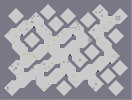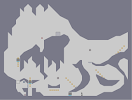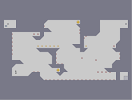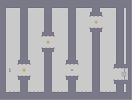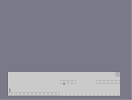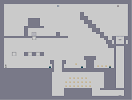Square race 2 or 3 (I don't remember) Stick to ground MULTI-MAP 2 [N-reality] Flappy N [N-reality] MULTI-MAP 3 [N-Reality] Ninja mario bros

Pages: (0)

wait a sec

are you a boy???
ok so how old are you and where are you from brother, you might be possibly guesssing who am i, i am Armaghan :D

it's worth mentioning
Ohh right okay that's cool man, I mean like I really have no clue what im doing when it comes to the technical stuff I wont lie! haha just appreciate good art man this be one of them
Love this detail of this, must have taken a fair amount of time!

Awesome!!

Though whats it mean by 792*600 image map?

been there done that

also here is actual in-game screenshot (the thumbnail is very wrong) http://puu.sh/c0KIU/2fc1f5e35f.png

LOL!

It seems to work! Does it change every ^792 to ^0, ^791 to ^1 etc...?

Wait, I didn't delete it.

I still have it. Have a look at the mirror image of this map :D
You will be AMAZED! :D
http://paste.droni.es/1993a78fe23c6af3c7cb8d3c [paste.droni.es]

Ya, I was excited at first too.

But, the program was of no real use. I tried mirroring some of my ddas but they were bugged and never ever a mirrored dda finished. :(
I was angry so I delelted it forever. (afaik)

I have seen one with 1 color

and it was a lot more buggy than this.

hey

wait

isn't that that program that turns images into N objects

isn't this generated

:v

damn nice.

also, that signature kinda thing in the corner - bitchin' in the fullsize.

You've trid programming too?!

Sounds fun. Does it also work on N-Reality maps?
I think N programs also win dronies, like kkstrong's Modmaster. You just earned a dronie I guess. :D
Really cool work Ors! I myself made a python program which could create mirror image of any map! ANY MAP! :D
Whow, that is way better than my crappy test thing. I wish i could actually vote on stuff, if i could this would instantly get a 5. Very nice work, even with the program used.